2018-07-04 20:08:23 gxc_8311 阅读数 1580
• ###### 3D游戏引擎之GPU渲染（DX篇）

12032 人正在学习 去看看 姜雪伟http://www.jikecloud.net/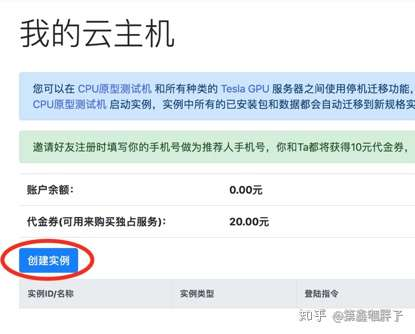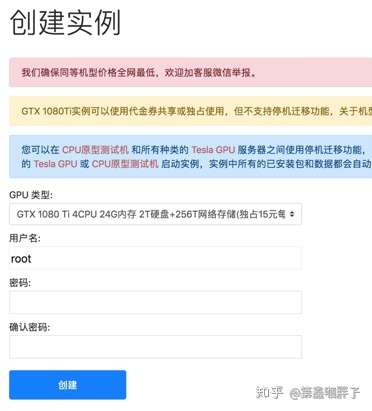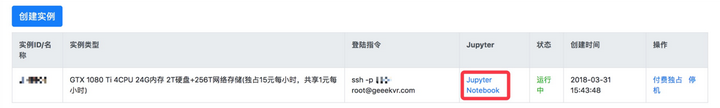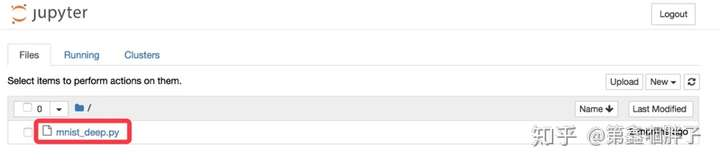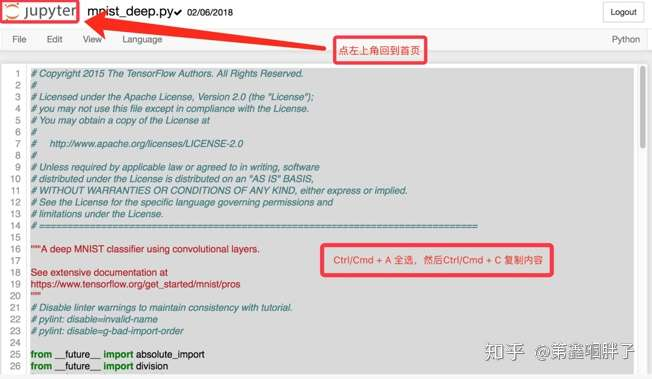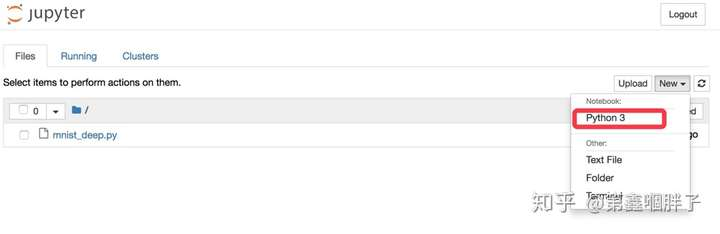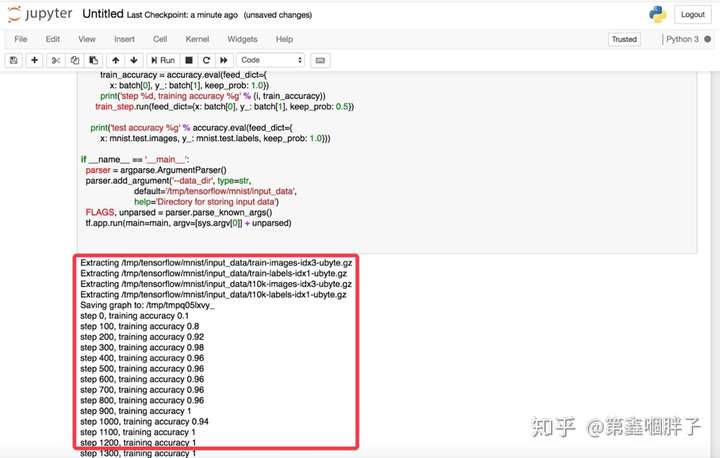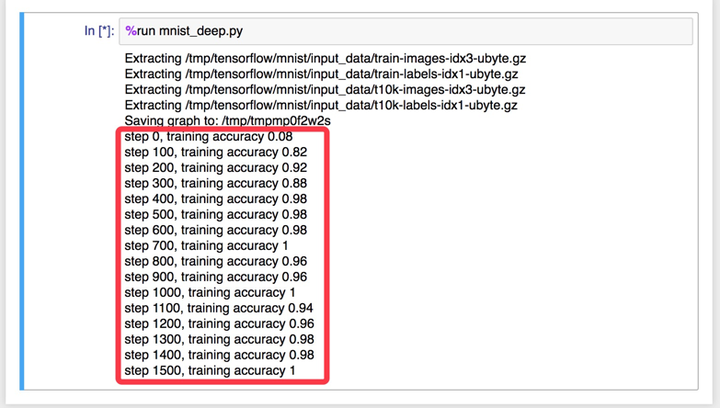2017-08-21 12:01:14 u010159842 阅读数 1068
• ###### 3D游戏引擎之GPU渲染（DX篇）

12032 人正在学习 去看看 姜雪伟

• 批量输入模块
• 各种深度学习零件搭建的深度神经网络
• 凸优化模块
• 模型的训练与评估

## 1. 结合传统数据处理方法的特征提取

from __future__ import print_function, division

import numpy as np
import os
import csv
from glob import glob
import pandas as pd
import numpy as np
import SimpleITK as sitk

from skimage import measure,morphology
from sklearn.cluster import KMeans
from skimage.transform import resize
import matplotlib.pyplot as plt
import seaborn as sns

from glob import glob

try:
from tqdm import tqdm # long waits are not fun
except:
print('TQDM does make much nicer wait bars...')
tqdm = lambda x: x

'''
Center : centers of circles px -- list of coordinates x,y,z
diam : diameters of circles px -- diameter
widthXheight : pixel dim of image
spacing = mm/px conversion rate np array x,y,z
origin = x,y,z mm np.array
z = z position of slice in world coordinates mm
'''
mask = np.zeros([height,width]) # 0's everywhere except nodule swapping x,y to match img
#convert to nodule space from world coordinates

# Defining the voxel range in which the nodule falls
v_center = (center-origin)/spacing
v_diam = int(diam/spacing+5)
v_xmin = np.max([0,int(v_center-v_diam)-5])
v_xmax = np.min([width-1,int(v_center+v_diam)+5])
v_ymin = np.max([0,int(v_center-v_diam)-5])
v_ymax = np.min([height-1,int(v_center+v_diam)+5])

v_xrange = range(v_xmin,v_xmax+1)
v_yrange = range(v_ymin,v_ymax+1)

# Convert back to world coordinates for distance calculation
x_data = [x*spacing+origin for x in range(width)]
y_data = [x*spacing+origin for x in range(height)]

# Fill in 1 within sphere around nodule
for v_x in v_xrange:
for v_y in v_yrange:
p_x = spacing*v_x + origin
p_y = spacing*v_y + origin
if np.linalg.norm(center-np.array([p_x,p_y,z]))<=diam:

def matrix2int16(matrix):
'''
matrix must be a numpy array NXN
Returns uint16 version
'''
m_min= np.min(matrix)
m_max= np.max(matrix)
matrix = matrix-m_min
return(np.array(np.rint( (matrix-m_min)/float(m_max-m_min) * 65535.0),dtype=np.uint16))

for fcount, img_file in enumerate(tqdm(df_node['seriesuid'])):
mini_df = df_node[df_node["seriesuid"]==img_file] #get all nodules associate with file
if mini_df.shape>0: # some files may not have a nodule--skipping those
img_array = sitk.GetArrayFromImage(itk_img) # indexes are z,y,x (notice the ordering)
num_z, height, width = img_array.shape        #heightXwidth constitute the transverse plane
origin = np.array(itk_img.GetOrigin())      # x,y,z  Origin in world coordinates (mm)
spacing = np.array(itk_img.GetSpacing())    # spacing of voxels in world coor. (mm)
# go through all nodes (why just the biggest?)
for node_idx, cur_row in mini_df.iterrows():
node_x = cur_row["coordX"]
node_y = cur_row["coordY"]
node_z = cur_row["coordZ"]
diam = cur_row["diameter_mm"]
# just keep 3 slices
imgs = np.ndarray([3,height,width],dtype=np.float32)
center = np.array([node_x, node_y, node_z])   # nodule center
v_center = np.rint((center-origin)/spacing)  # nodule center in voxel space (still x,y,z ordering)
for i, i_z in enumerate(np.arange(int(v_center)-1,
int(v_center)+2).clip(0, num_z-1)): # clip prevents going out of bounds in Z
width, height, spacing, origin)
imgs[i] = img_array[i_z]
np.save(os.path.join("./images_%04d_%04d.npy" % (fcount,node_idx)),imgs)


x seriesuid coordX coordY coordZ diameter_mm
0 1.3.6.1.4.1.14519.5.2.1.6279.6001.179049373636... 56.208405 86.343413 -115.867579 23.350644

img_file = "./images_0000_0000.npy"

fig = plt.figure(figsize=(12,4))
for i in range(3):
ax.imshow(imgs_to_process[i,:,:], 'bone')
ax.set_axis_off()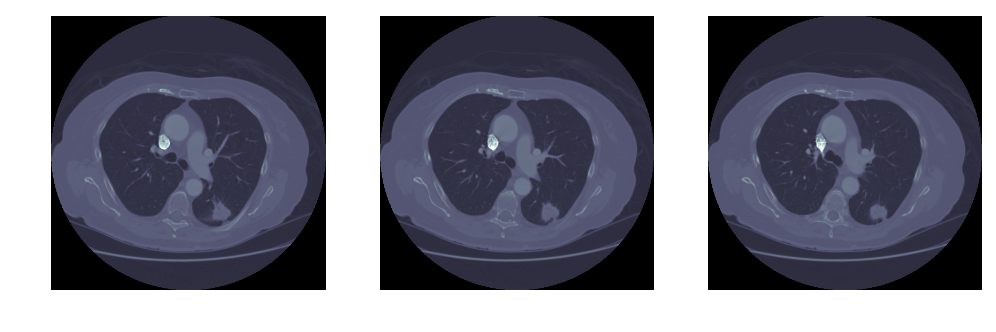i = 0
img = imgs_to_process[i]

#Standardize the pixel values
mean = np.mean(img)
std = np.std(img)
img = img-mean
img = img/std

# Find the average pixel value near the lungs
#         to renormalize washed out images
middle = img[100:400,100:400]
mean = np.mean(middle)
max = np.max(img)
min = np.min(img)

# To improve threshold finding, I'm moving the
#         underflow and overflow on the pixel spectrum
img[img==max]=mean
img[img==min]=mean

# Using Kmeans to separate foreground (radio-opaque tissue)
#     and background (radio transparent tissue ie lungs)
# Doing this only on the center of the image to avoid
#     the non-tissue parts of the image as much as possible
kmeans = KMeans(n_clusters=2).fit(np.reshape(middle,[np.prod(middle.shape),1]))
centers = sorted(kmeans.cluster_centers_.flatten())
threshold = np.mean(centers)
thresh_img = np.where(img<threshold,1.0,0.0)  # threshold the image


# 对一张图中所有像素点的亮度做概率密度分布, 用竖线标注阈值所在
fig = plt.figure(figsize=(8,12))
sns.distplot(middle.ravel(), ax=ax1)
ax1.vlines(x=threshold, ymax=10, ymin=0)
ax1.set_title('Threshold: %1.2F' % threshold)
ax1.set_xticklabels([])

# 展示阈值对图像切割的结果。小于阈值的点标注为1，白色。大于阈值的点标注为0，黑色。
ax2.imshow(thresh_img, "gray")
ax2.set_axis_off()
ax2.set_title('Step1, using threshold as cutoff')

# 增大黑色部分（非ROI）的区域，使之尽可能的连在一起
eroded = morphology.erosion(thresh_img,np.ones([4,4]))
ax3.imshow(eroded, "gray")
ax3.set_axis_off()
ax3.set_title('Step2,  erosion shrinks bright\nregions and enlarges dark regions.')

# 增大白色部分（ROI）的区域，尽可能的消除面积较小的黑色区域
dilation = morphology.dilation(eroded,np.ones([10,10]))
ax4.imshow(dilation, "gray")
ax4.set_axis_off()
ax4.set_title('Step3,  dilation shrinks dark\nregions and enlarges bright regions.')

# 上一张图中共有三片连续区域，即最外层的体外区域，内部的肺部区域，以及二者之间的身体轮廓区域。这里将其分别标出
labels = measure.label(dilation)
ax5.imshow(labels, "gray")
#ax5.set_axis_off()
ax5.set_title('Step4, label connected regions\n of an integer array.')

# 提取regions 信息，这张图片的 region的 bbox位置分别在 [[0,0,512,512],[141, 86, 396, 404]],
#   分别对应 体外+轮廓 以及 肺部区域的左上角、右下角坐标。
#   于是这里通过区域的宽度 B-B、高度 B-B
#   以及距离图片上下的距离  B>40 and B<472,
#   最终保留需要的区域。
regions = measure.regionprops(labels)
good_labels = []
for prop in regions:
B = prop.bbox
if B-B<475 and B-B<475 and B>40 and B<472:
good_labels.append(prop.label)

for N in good_labels:

ax6.set_axis_off()
ax6.set_title('Step5, remain the region of interests.')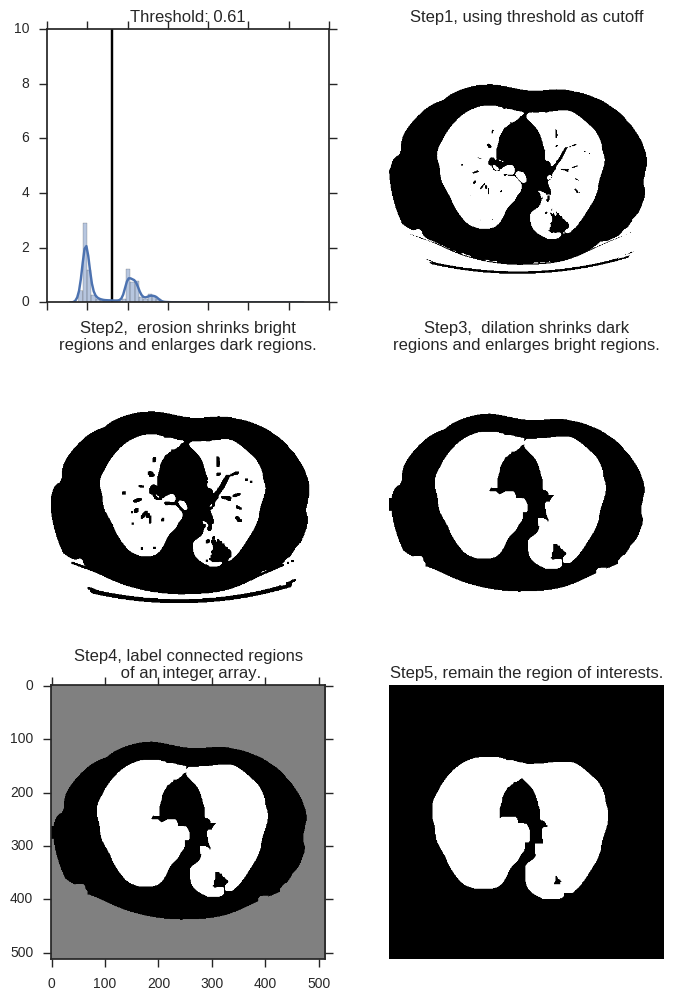fig = plt.figure(figsize=(8,4))

ax1.imshow(imgs_to_process[0,:,:], 'bone')
ax1.set_axis_off()
ax1.set_title("Raw CT image")

ax2.set_axis_off()
ax2.set_title("Pre-processed Images with\nregion of interest in lung")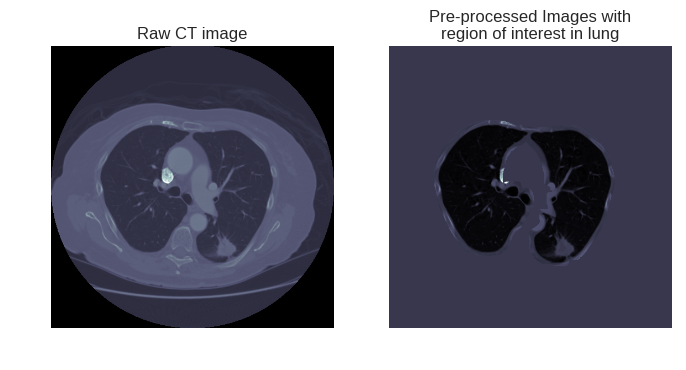## 2. 结合深度学习技术的特征提取增强wget https://github.com/daviddao/spatial-transformer-tensorflow/raw/master/data/mnist_sequence1_sample_5distortions5x5.npzimport tensorflow as tf
# https://github.com/tensorflow/models/tree/master/transformer
from spatial_transformer import transformer
import numpy as np
from tf_utils import weight_variable, bias_variable, dense_to_one_hot
import matplotlib.pyplot as plt
from keras.backend.tensorflow_backend import set_session

np.random.seed(0)
tf.set_random_seed(0)

config = tf.ConfigProto()
config.gpu_options.allow_growth=True
set_session(tf.Session(config=config))
%matplotlib inline

X_train = mnist_cluttered['X_train']
y_train = mnist_cluttered['y_train']
X_valid = mnist_cluttered['X_valid']
y_valid = mnist_cluttered['y_valid']
X_test = mnist_cluttered['X_test']
y_test = mnist_cluttered['y_test']

Y_train = dense_to_one_hot(y_train, n_classes=10)
Y_valid = dense_to_one_hot(y_valid, n_classes=10)
Y_test = dense_to_one_hot(y_test, n_classes=10)


x = tf.placeholder(tf.float32, [None, 1600])
keep_prob = tf.placeholder(tf.float32)

iter_per_epoch = 100
n_epochs = 500
train_size = 10000
indices = np.linspace(0, 10000 - 1, iter_per_epoch)
indices = indices.astype('int')
iter_i = 0

batch_xs = X_train[indices[iter_i]:indices[iter_i+1]]
x_tensor = tf.reshape(x, [-1, 40, 40, 1])

sess = tf.Session()
sess.run(tf.global_variables_initializer())
xout = sess.run(x_tensor, feed_dict={x: batch_xs})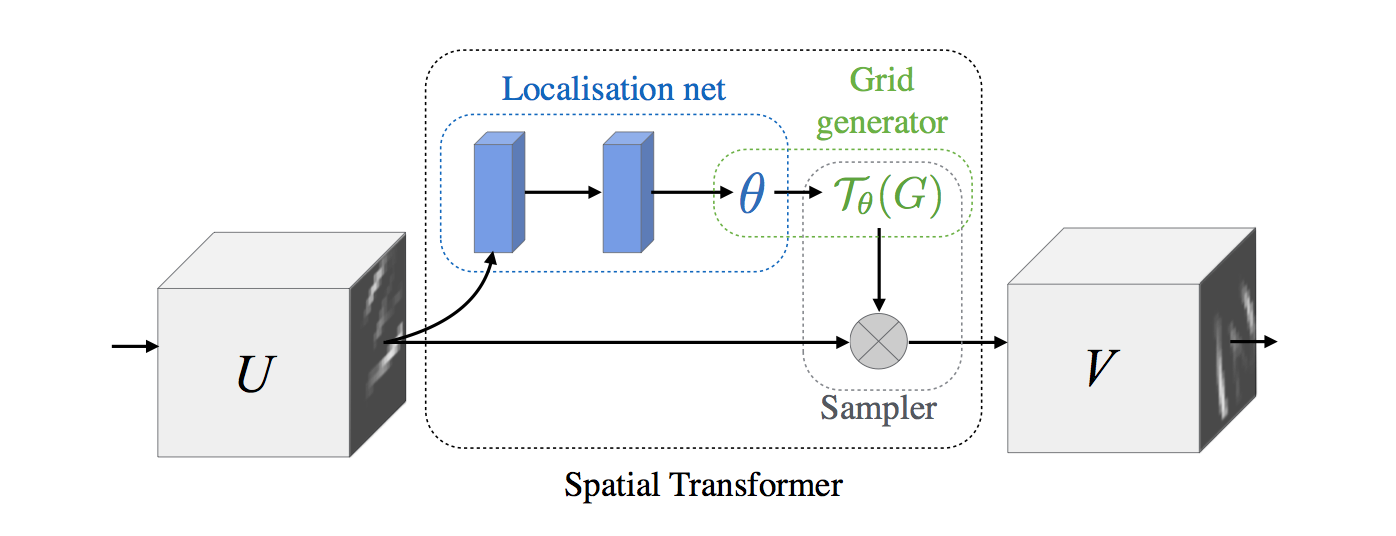x = tf.placeholder(tf.float32, [None, 1600])
y = tf.placeholder(tf.float32, [None, 10])

x_tensor = tf.reshape(x, [-1, 40, 40, 1])
W_fc_loc1 = weight_variable([1600, 20])
b_fc_loc1 = bias_variable()

W_fc_loc2 = weight_variable([20, 6])
initial = np.array([[1., 0, 0], [0, 1., 0]])
initial = initial.astype('float32')
initial = initial.flatten()
b_fc_loc2 = tf.Variable(initial_value=initial, name='b_fc_loc2')

# %% Define the two layer localisation network
h_fc_loc1 = tf.nn.tanh(tf.matmul(x, W_fc_loc1) + b_fc_loc1)

# %% We can add dropout for regularizing and to reduce overfitting like so:
keep_prob = tf.placeholder(tf.float32)
h_fc_loc1_drop = tf.nn.dropout(h_fc_loc1, keep_prob)

# %% Second layer
h_fc_loc2 = tf.nn.tanh(tf.matmul(h_fc_loc1_drop, W_fc_loc2) + b_fc_loc2)

# %% We'll create a spatial transformer module to identify discriminative
# %% patches
out_size = (40, 40)
h_trans = transformer(x_tensor, h_fc_loc2, out_size)


iter_i = 0
batch_xs = X_train[0:101]
batch_ys = Y_train[0:101]

sess = tf.Session()
sess.run(tf.global_variables_initializer())

xtransOut = sess.run(h_trans,
feed_dict={
x: batch_xs,
y: batch_ys,
keep_prob: 1.0
})


fig = plt.figure(figsize=(10,2))

for idx in range(10):

ax1.imshow(xout[idx,:,:,0], "gray")
ax2.imshow(xtransOut[idx,:,:,0], "gray")
ax1.set_axis_off()
ax2.set_axis_off()
ax1.set_title(np.argmax(batch_ys, axis=1)[idx])
ax2.set_title(np.argmax(batch_ys, axis=1)[idx])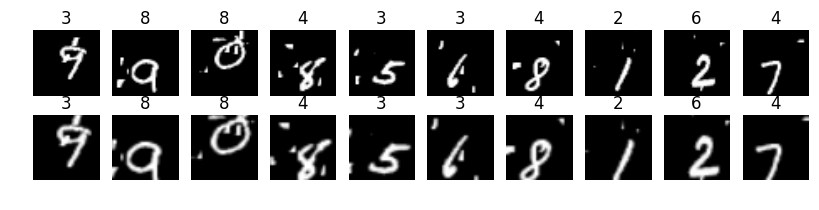$T_{\theta(G)}$ ，可以将一个倾斜的、“草书”书写的数字，变得更正一点。x = tf.placeholder(tf.float32, [None, 1600])
y = tf.placeholder(tf.float32, [None, 10])
keep_prob = tf.placeholder(tf.float32)

def Networks(x,keep_prob, SpatialTrans=True):
x_tensor = tf.reshape(x, [-1, 40, 40, 1])
W_fc_loc1 = weight_variable([1600, 20])
b_fc_loc1 = bias_variable()

W_fc_loc2 = weight_variable([20, 6])
initial = np.array([[1., 0, 0], [0, 1., 0]])
initial = initial.astype('float32')
initial = initial.flatten()
b_fc_loc2 = tf.Variable(initial_value=initial, name='b_fc_loc2')

# %% Define the two layer localisation network
h_fc_loc1 = tf.nn.tanh(tf.matmul(x, W_fc_loc1) + b_fc_loc1)
# %% We can add dropout for regularizing and to reduce overfitting like so:

h_fc_loc1_drop = tf.nn.dropout(h_fc_loc1, keep_prob)
# %% Second layer
h_fc_loc2 = tf.nn.tanh(tf.matmul(h_fc_loc1_drop, W_fc_loc2) + b_fc_loc2)

# %% We'll create a spatial transformer module to identify discriminative
# %% patches
out_size = (40, 40)
h_trans = transformer(x_tensor, h_fc_loc2, out_size)

# %% We'll setup the first convolutional layer
# Weight matrix is [height x width x input_channels x output_channels]
filter_size = 3
n_filters_1 = 16
W_conv1 = weight_variable([filter_size, filter_size, 1, n_filters_1])

# %% Bias is [output_channels]
b_conv1 = bias_variable([n_filters_1])

# %% Now we can build a graph which does the first layer of convolution:
# we define our stride as batch x height x width x channels
# instead of pooling, we use strides of 2 and more layers
# with smaller filters.
if SpatialTrans:
h_conv1 = tf.nn.relu(
tf.nn.conv2d(input=h_trans,
filter=W_conv1,
strides=[1, 2, 2, 1],
b_conv1)
else:
h_conv1 = tf.nn.relu(
tf.nn.conv2d(input=x_tensor,
filter=W_conv1,
strides=[1, 2, 2, 1],
b_conv1)

# %% And just like the first layer, add additional layers to create
# a deep net
n_filters_2 = 16
W_conv2 = weight_variable([filter_size, filter_size, n_filters_1, n_filters_2])
b_conv2 = bias_variable([n_filters_2])
h_conv2 = tf.nn.relu(
tf.nn.conv2d(input=h_conv1,
filter=W_conv2,
strides=[1, 2, 2, 1],
b_conv2)

# %% We'll now reshape so we can connect to a fully-connected layer:
h_conv2_flat = tf.reshape(h_conv2, [-1, 10 * 10 * n_filters_2])

# %% Create a fully-connected layer:
n_fc = 1024
W_fc1 = weight_variable([10 * 10 * n_filters_2, n_fc])
b_fc1 = bias_variable([n_fc])
h_fc1 = tf.nn.relu(tf.matmul(h_conv2_flat, W_fc1) + b_fc1)

h_fc1_drop = tf.nn.dropout(h_fc1, keep_prob)

# %% And finally our softmax layer:
W_fc2 = weight_variable([n_fc, 10])
b_fc2 = bias_variable()
y_logits = tf.matmul(h_fc1_drop, W_fc2) + b_fc2
return y_logits

# %% We'll now train in minibatches and report accuracy, loss:
iter_per_epoch = 100
n_epochs = 100
train_size = 10000

indices = np.linspace(0, 10000 - 1, iter_per_epoch)
indices = indices.astype('int')


y_logits_F = Networks(x, keep_prob, SpatialTrans=False)
cross_entropy_F = tf.reduce_mean(tf.nn.softmax_cross_entropy_with_logits(logits=y_logits_F, labels=y))
optimizer_F = opt.minimize(cross_entropy_F)
correct_prediction_F = tf.equal(tf.argmax(y_logits_F, 1), tf.argmax(y, 1))
accuracy_F = tf.reduce_mean(tf.cast(correct_prediction_F, 'float'))

sessF = tf.Session()
sessF.run(tf.global_variables_initializer())

l_acc_F = []
for epoch_i in range(n_epochs):
for iter_i in range(iter_per_epoch - 1):
batch_xs = X_train[indices[iter_i]:indices[iter_i+1]]
batch_ys = Y_train[indices[iter_i]:indices[iter_i+1]]
sessF.run(optimizer_F, feed_dict={
x: batch_xs, y: batch_ys, keep_prob: 0.8})

acc = sessF.run(accuracy_F,
feed_dict={
x: X_valid,
y: Y_valid,
keep_prob: 1.0
})
l_acc_F.append(acc)
if epoch_i % 10 == 0:
print('Accuracy (%d): ' % epoch_i + str(acc))

Accuracy (0): 0.151
Accuracy (10): 0.813
Accuracy (20): 0.832
Accuracy (30): 0.825
Accuracy (40): 0.833
Accuracy (50): 0.837
Accuracy (60): 0.832
Accuracy (70): 0.837
Accuracy (80): 0.833
Accuracy (90): 0.843


y_logits = Networks(x, keep_prob)
cross_entropy = tf.reduce_mean(tf.nn.softmax_cross_entropy_with_logits(logits=y_logits, labels=y))
optimizer = opt.minimize(cross_entropy)
correct_prediction = tf.equal(tf.argmax(y_logits, 1), tf.argmax(y, 1))
accuracy = tf.reduce_mean(tf.cast(correct_prediction, 'float'))

sess = tf.Session()
sess.run(tf.global_variables_initializer())

l_acc = []
for epoch_i in range(n_epochs):
for iter_i in range(iter_per_epoch - 1):
batch_xs = X_train[indices[iter_i]:indices[iter_i+1]]
batch_ys = Y_train[indices[iter_i]:indices[iter_i+1]]
sess.run(optimizer, feed_dict={
x: batch_xs, y: batch_ys, keep_prob: 0.8})

acc = sess.run(accuracy,
feed_dict={
x: X_valid,
y: Y_valid,
keep_prob: 1.0
})
l_acc.append(acc)
if epoch_i % 10 == 0:
print('Accuracy (%d): ' % epoch_i + str(acc))


Accuracy (0): 0.25
Accuracy (10): 0.92
Accuracy (20): 0.94
Accuracy (30): 0.955
Accuracy (40): 0.943
Accuracy (50): 0.944
Accuracy (60): 0.957
Accuracy (70): 0.948
Accuracy (80): 0.941
Accuracy (90): 0.948


plt.plot(l_acc, label="Using Spatial Transform")
plt.plot(l_acc_F, label="Raw input")
plt.legend(loc=8)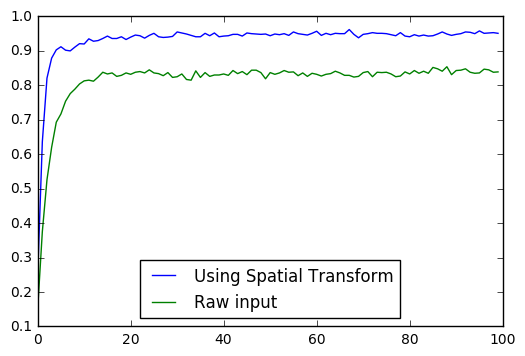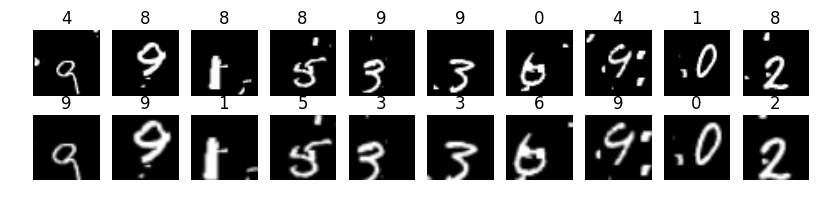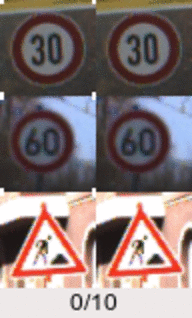2020-04-03 01:45:27 weixin_42588541 阅读数 9
• ###### 3D游戏引擎之GPU渲染（DX篇）

12032 人正在学习 去看看 姜雪伟

## 前言

最近参加计算机视觉相关的比赛，发现笔记本的GPU已经无法承受庞大数据集的摧残，转而使用云GPU来跑代码，由于对Linux系统的不熟悉反复出了很多问题，至今终于配制好环境代码可以正常运行了，特此记录：

## 配置记录

在查找资料时常常会找到很多过时或者不符合自己情况的代码，在自己写文的时候就把这些内容标清楚了，如果配置不同可以先弄清楚是否可迁移再运行

配置 版本
操作系统 Ubuntu 16.04.6 LTS 4.13.0-36-generic GNU/Linux
python 3.6.3
cuda 10.0

## 详细步骤

我需求的环境为python == 3.6，pytorch == 1.1.0，缝缝补补，在看了很多网站之后，以下操作已经能够满足我的需求配置好环境，下面会将所有的信息来源和代码标示出来，需求不同的朋友可以做个借鉴，调整自己的设置

### 一、安装依赖

sudo apt-get install python-dev
sudo apt-get install libffi-dev
sudo apt-get install libssl-dev
sudo apt-get install libxml2-dev
sudo apt-get install libxslt-dev
sudo apt-get install libsqlite3-dev
sudo apt-get install zlib1g-dev
sudo apt-get install libbz2-dev


### 二、安装python 3.6.3包

cd /opt
wget https://www.python.org/ftp/python/3.6.3/Python-3.6.3.tgz
tar -xvf Python-3.6.3.tgz
cd Python-3.6.3
./configure
make
make install
update-alternatives --install /usr/bin/python3 python3 /usr/bin/python3.5 1
update-alternatives --install /usr/bin/python3 python3 /usr/local/bin/python3.6 2
update-alternatives --config python3
mv /usr/bin/python /usr/bin/python.bak
ln -s /usr/local/bin/python3 /usr/bin/python


### 三、安装pip

sudo rm /usr/bin/lsb_release
curl https://bootstrap.pypa.io/get-pip.py -o get-pip.py
python3 get-pip.py --force-reinstall


### 四、更新jupyterlab内核

pip3 install ipykernel
python -m ipykernel install


### 五、总结

环境配置比较繁琐，但接下来要学习的东西还有更多更多，写下这些作为记录，也希望能帮到正在苦苦debug的你

云gpu 深度学习 相关内容

2018-04-26 11:40:38 yunqiinsight 阅读数 610
• ###### 3D游戏引擎之GPU渲染（DX篇）

12032 人正在学习 去看看 姜雪伟

摘要： 本文根据实测数据，初步探讨了在弹性GPU云服务器上深度学习的性能模型，可帮助科学选择GPU实例的规格。

1 背景

得益于GPU强大的计算能力，深度学习近年来在图像处理、语音识别、自然语言处理等领域取得了重大突破，GPU服务器几乎成了深度学习加速的标配。
阿里云GPU云服务器在公有云上提供的弹性GPU服务，可以帮助用户快速用上GPU加速服务，并大大简化部署和运维的复杂度。如何提供一个合适的实例规格，从而以最高的性价比提供给深度学习客户，是我们需要考虑的一个问题，本文试图从CPU、内存、磁盘这三个角度对单机GPU云服务器的深度学习训练和预测的性能模型做了初步的分析，希望能对实例规格的选择提供一个科学的设计模型。
下面是我们使用主流的几个开源深度学习框架在NVIDIA GPU上做的一些深度学习的测试。涉及NVCaffe、MXNet主流深度学习框架，测试了多个经典CNN网络在图像分类领域的训练和推理以及RNN网络在自然语言处理领域的训练。

2 训练测试

我们使用NVCaffe、MXNet主流深度学习框架测试了图像分类领域和自然语言处理领域的训练模型。

2.1 图像分类
我们使用NVCaffe、MXNet测试了图像分类领域的CNN网络的单GPU模型训练。
NVCaffe和MXNet测试使用ImageNet ILSVRC2012数据集，训练图片1281167张，包含1000个分类，每个分类包含1000张左右的图片。

2.1.1 CPU+Memory

2.1.1.1 NVCaffe

NVCaffe是NVIDIA基于BVLC-Caffe针对NVIDIA GPU尤其是多GPU加速的开源深度学习框架。LMDB格式的ImageNet训练集大小为240GB ，验证集大小为9.4GB。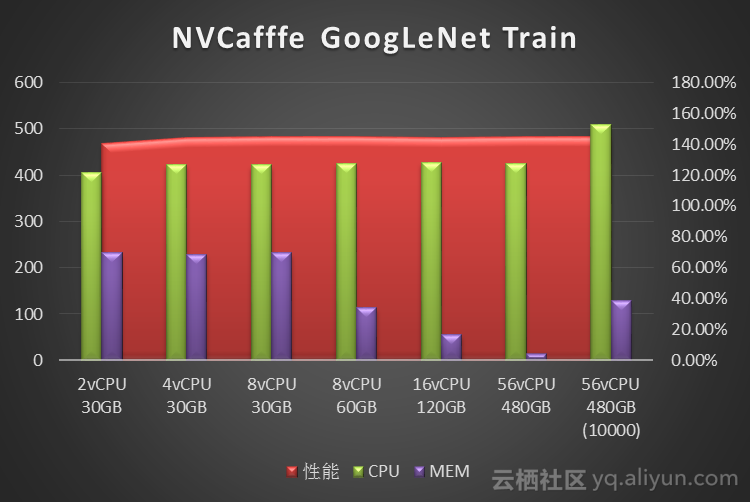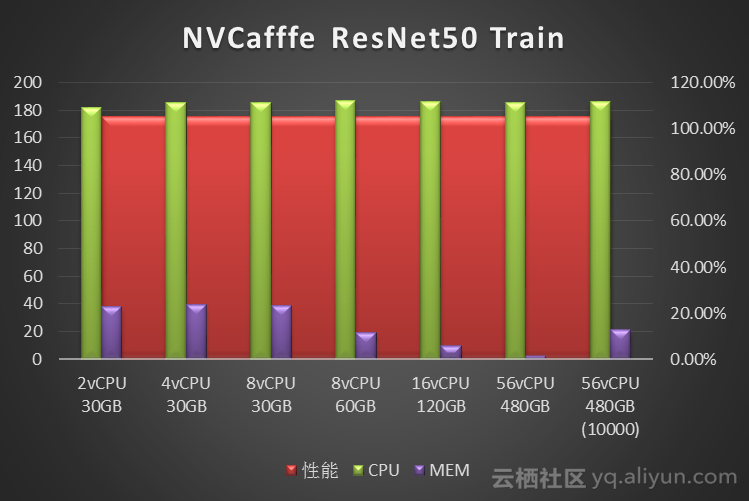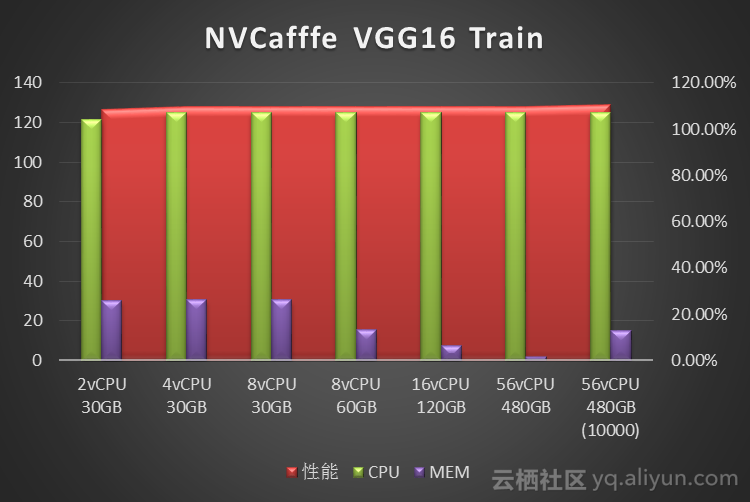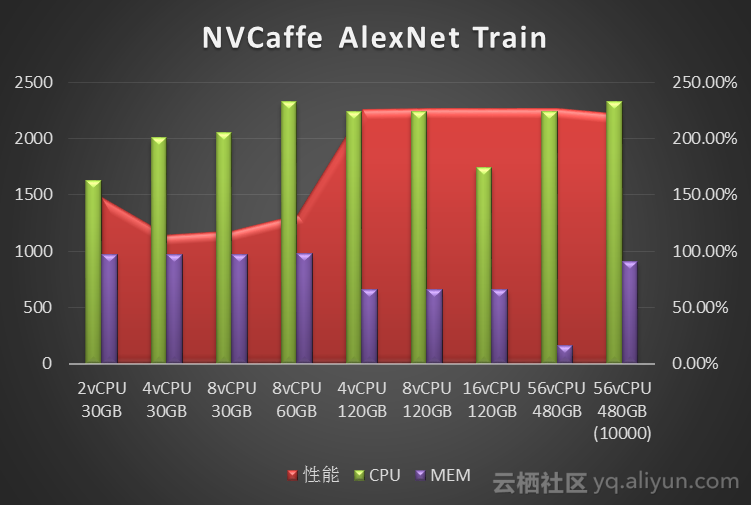2.1.1.2 MXNet
MXNet的数据集使用RecordIO格式，ImageNet训练集 93GB ，验证集 3.7GB。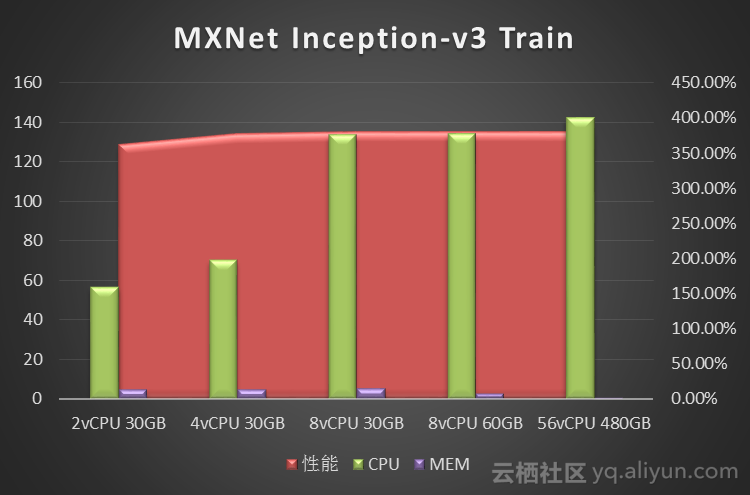2.1.2 磁盘IO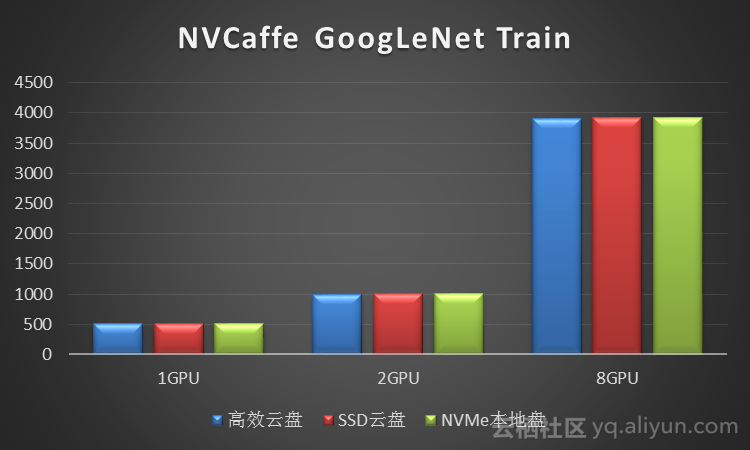2.2 自然语言处理
我们使用MXNet测试了RNN网络的LSTM模型的训练，使用PennTreeBank自然语言数据集。PennTreeBank数据集的文本语料库包含近100万个单词，单词表被限定在10000个单词。分别对比了不同vCPU和Memory配置下的训练性能：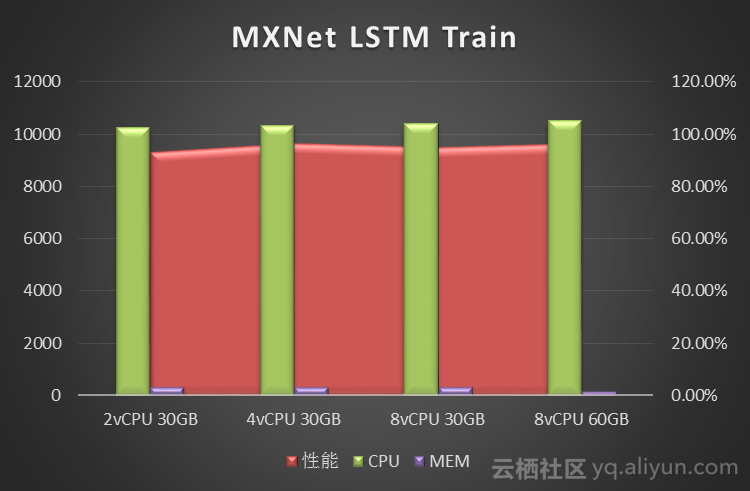3 推理测试

3.1 图像分类

我们使用NVCaffe测试了图像分类领域的CNN网络的模型推理。
测试使用ImageNet ILSVRC2012数据集，验证测试图片 50000张。

3.1.1 CPU+Memory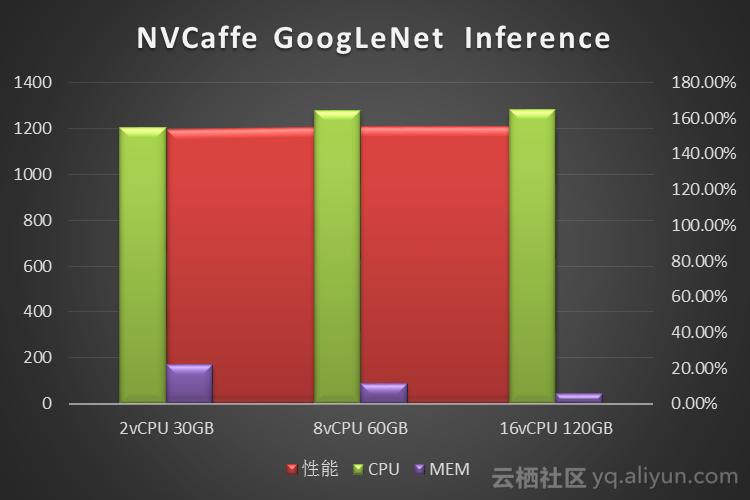3.1.2 磁盘IO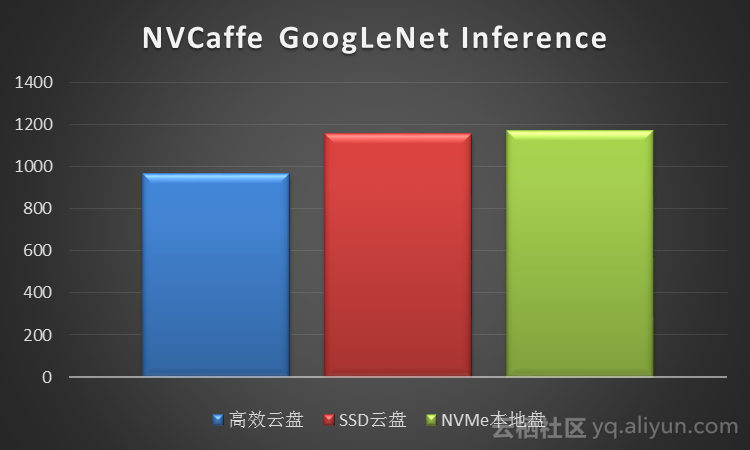4 数据预处理测试

在训练模型之前，往往要对训练数据集做数据预处理，统一数据格式，并做一定的归一化处理。
我们使用NVCaffe对ImageNet ILSVRC2012数据集做了数据预处理的测试，分别对比了NVMe SSD本地盘、SSD云盘和高效云盘的数据预处理时间，数据单位是秒，数据如下：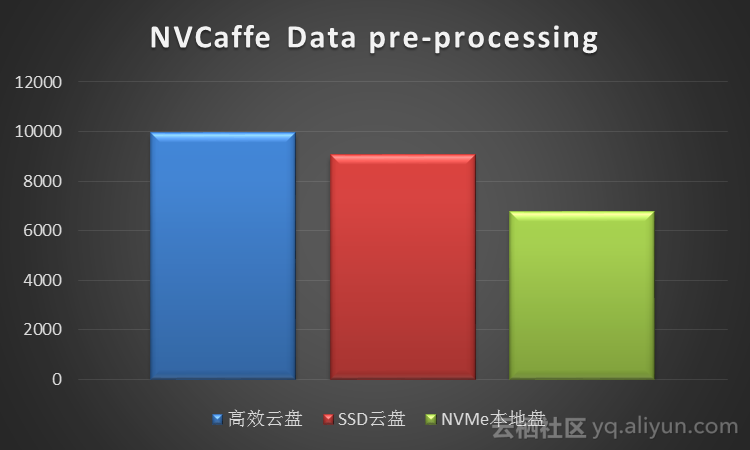5 数据分析

5.1 训练

5.1.1 图像分类
从NVCaffe和MXNet的测试结果来看，图像分类场景单纯的训练阶段对CPU要求不高，单GPU 只需要4vCPU就可以。而内存需求则取决于深度学习框架、神经网络类型和训练数据集的大小：测试中发现NVCaffe随着迭代次数的增多，内存是不断增大的，但是内存需求增大到一定程度，对性能就不会有什么提升了，其中NVCaffe AlexNet网络的训练，相比其它网络对于内存的消耗要大得多。相比之下MXNet的内存占用则要小的多（这也是MXNet的一大优势），93G预处理过的训练数据集训练过程中内存占用不到5G。
对于磁盘IO性能，测试显示训练阶段NVMe SSD本地盘、SSD云盘性能基本接近，高效云盘上的性能略差1%。因此训练阶段对IO性能的要求不高。

5.1.2 自然语言处理
从MXNet的测试结果来看，对于PennTreeBank这样规模的数据集，2vCPU 1GB Mem就能满足训练需求。由于自然语言处理的原始数据不像图像分类一样是大量高清图片，自然语言处理的原始数据以文本文件为主，因此自然语言处理对内存和显存的要求都不高，从我们的测试来看，4vCPU 30GB 1GPU规格基本满足训练阶段需求。

5.2 推理

5.2.1 图像分类
从NVCaffe的图像分类推理测试来看，除AlexNet 2vCPU刚刚够用外，其它网络2vCPU对性能没有影响，而9.4GB的验证数据集推理过程中内存占用大概是7GB左右，因此对大部分模型来看，2vCPU 30GB 1GPU规格基本满足图像分类推理的性能需求。
对于磁盘IO性能，推理性能NVMe SSD本地盘、SSD云盘很接近，但高效云盘差15%。因此推理阶段至少应该使用SSD云盘保证性能。
5.2.2 自然语言处理
对于自然语言处理，参考训练性能需求，我们应该可以推测2vCPU 30GB 1GPU规格应该也能满足需求。

5.3 数据预处理

从NVCaffe对ImageNet ILSVRC2012数据集做数据预处理的测试来看，数据预处理阶段是IO密集型，NVMe SSD本地盘比SSD云盘快25%，而SSD云盘比高效云盘快10%。

6 总结

深度学习框架众多，神经网络类型也是种类繁多，我们选取了主流的框架和神经网络类型，尝试对单机GPU云服务器的深度学习性能模型做了初步的分析，结论是：

1. 深度学习训练阶段是GPU运算密集型，对于CPU占用不大，而内存的需求取决于深度学习框架、神经网络类型和训练数据集的大小；对磁盘IO性能不敏感，云盘基本能够满足需求。
2. 深度学习推理阶段对于CPU的占用更小，但是对于磁盘IO性能相对较敏感，因为推理阶段对于延迟有一定的要求，更高的磁盘IO性能对于降低数据读取的延时进而降低整体延迟有很大的帮助。
3. 深度学习数据预处理阶段是IO密集型阶段，更高的磁盘IO性能能够大大缩短数据预处理的时间。

原文链接

阅读更多干货好文，请关注扫描以下二维码：云gpu 深度学习 相关内容

2020-03-02 00:17:07 CZW199418 阅读数 240
• ###### 3D游戏引擎之GPU渲染（DX篇）

12032 人正在学习 去看看 姜雪伟

## 1.创建云服务器

### 1.1注册滴滴云

首先注册滴滴云，并进行实名认证，否则无法创建云服务器。点击“控制台”，进入控制台页面。

### 1.2选择配置

点击快速创建云服务器(DC2)，显示出云服务器配置列表。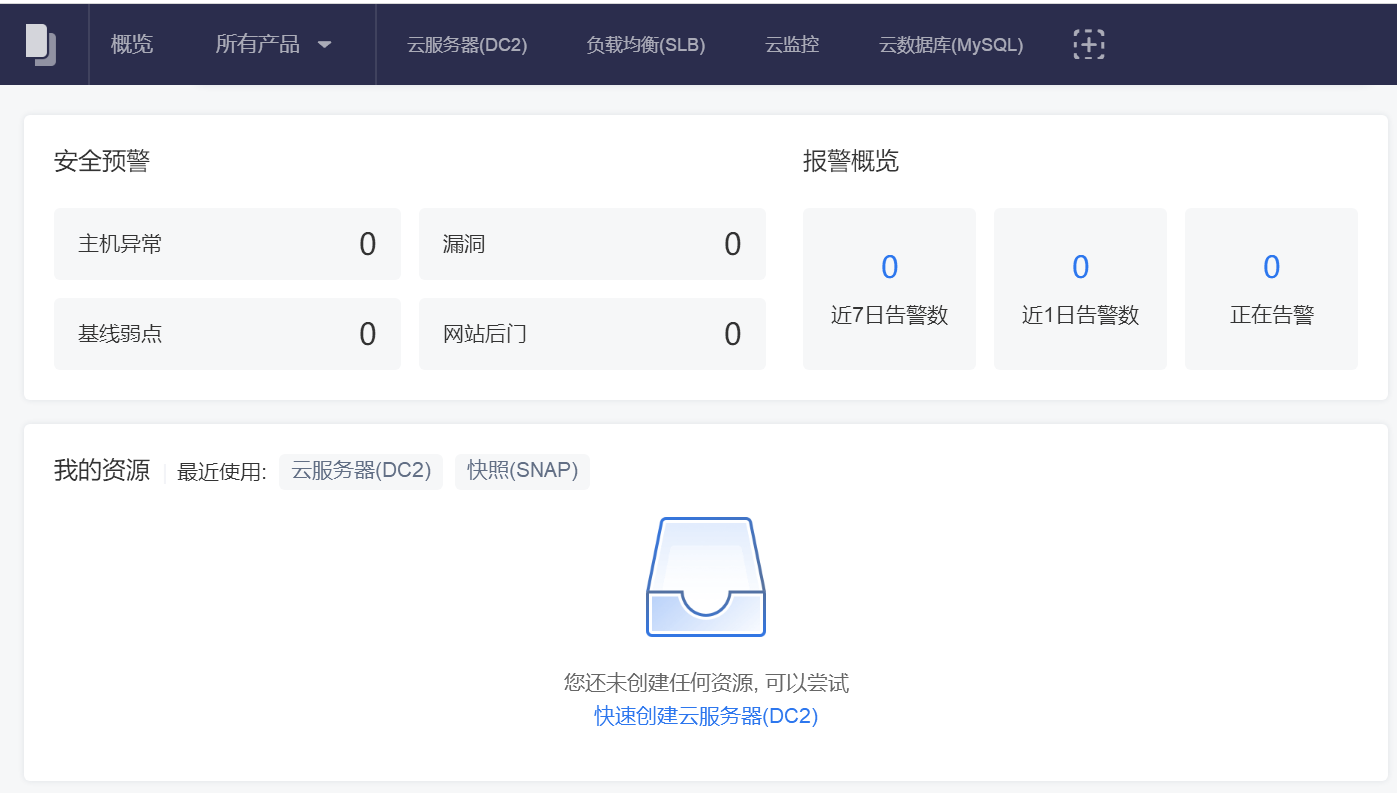云服务器配置选择：

付费方式：按时长。包月价格过高。

可用区：广东1区。理论上北京区更好，但是北京区GPU服务器太差。

服务器类型：GPU云服务器，通用型P100。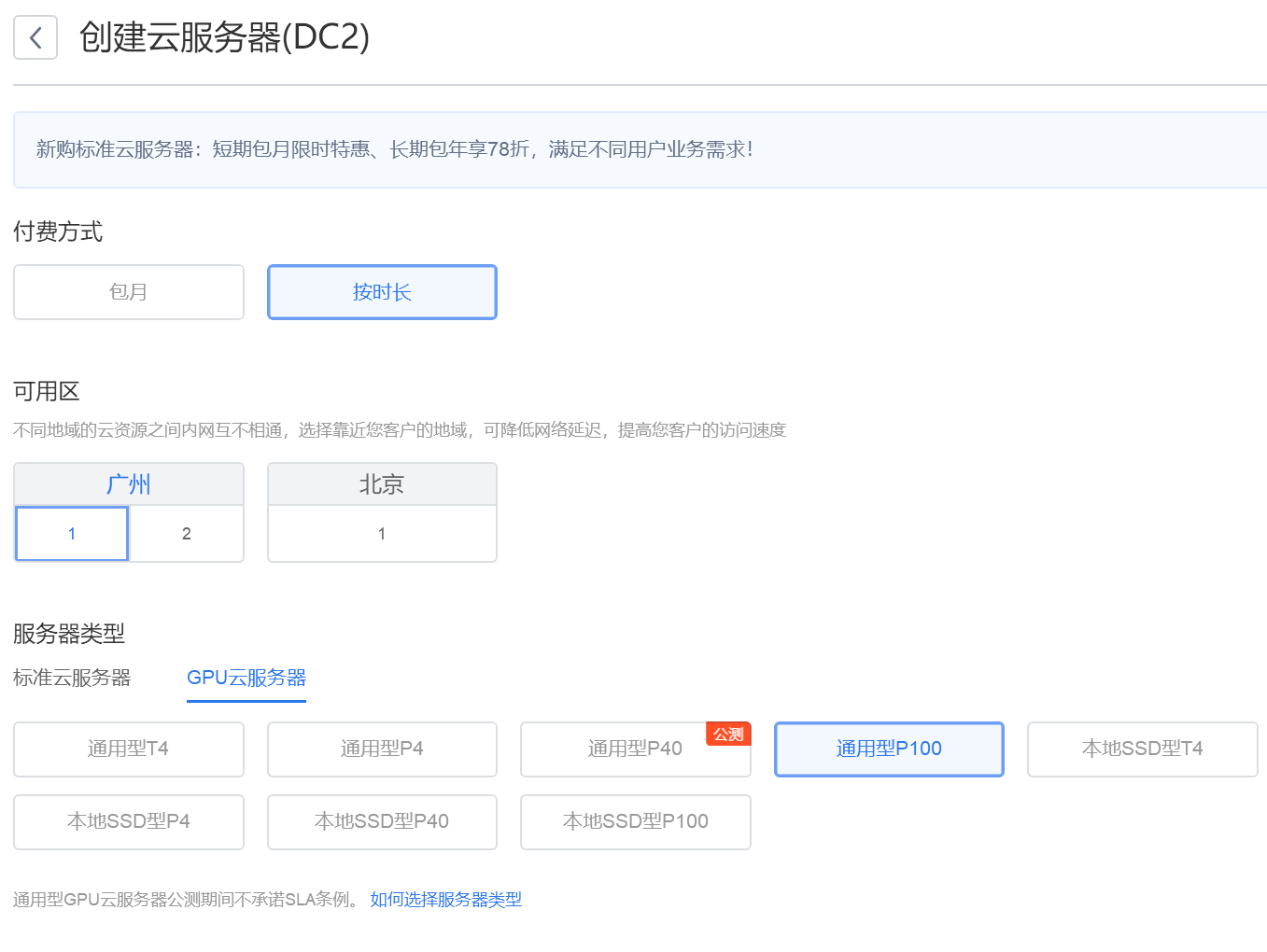镜像：标准镜像，这里我选择已经安装好CUDA的版本。

配置：根据需要选择，一般选择第一个单GPU即可。

系统盘和数据盘根据自己需要调整。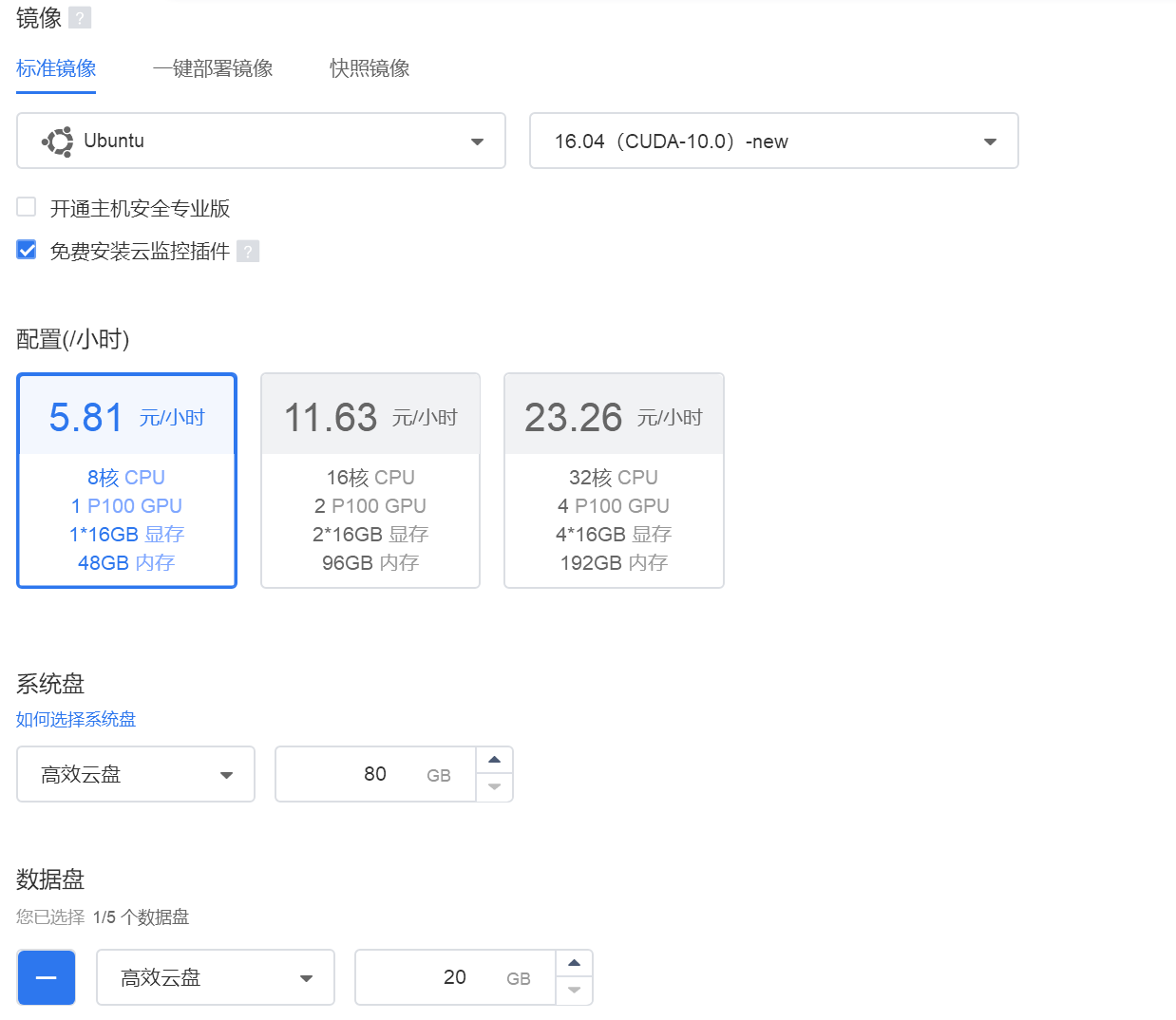网络按照默认即可，宽带选择10M，基本上够用，安全组按照默认。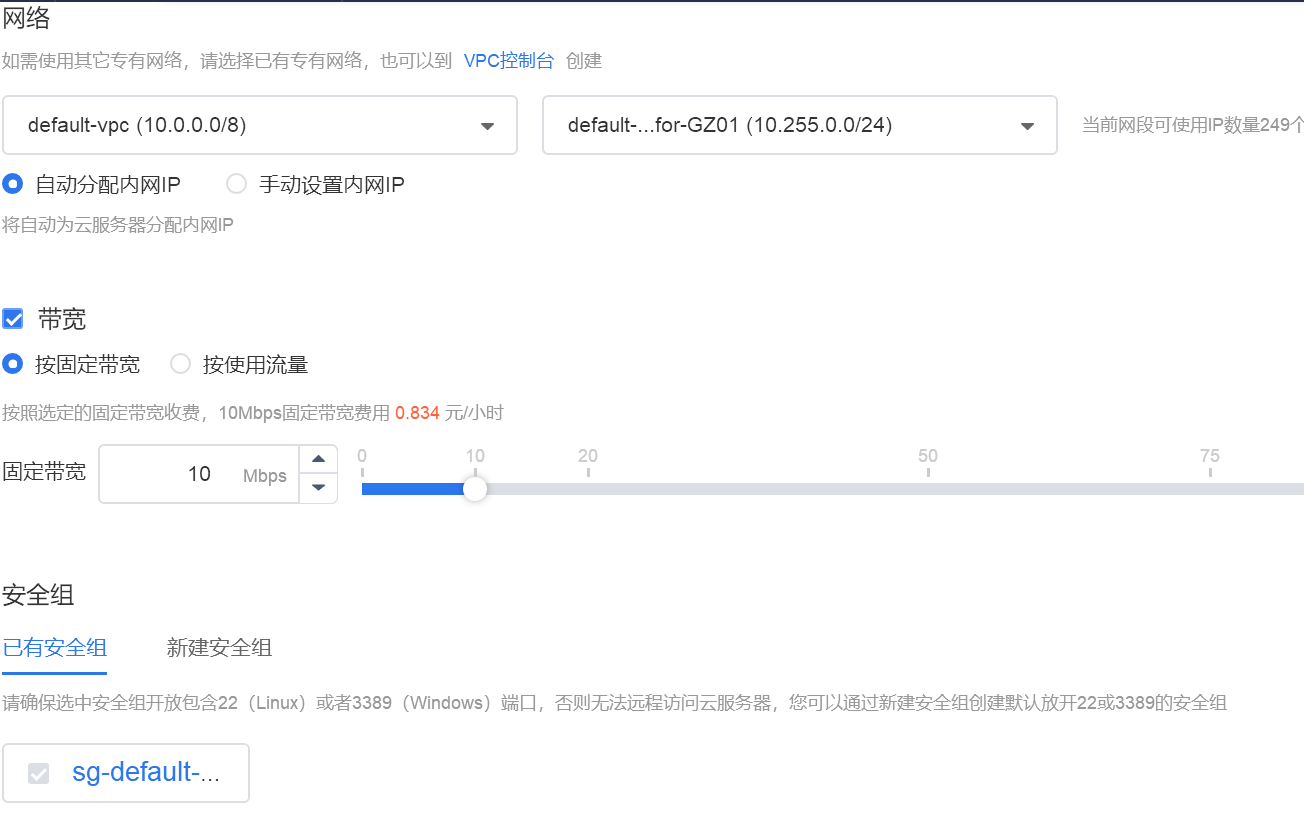登陆方式选择密码，其他按照默认即可，添加标签，也可以不添加。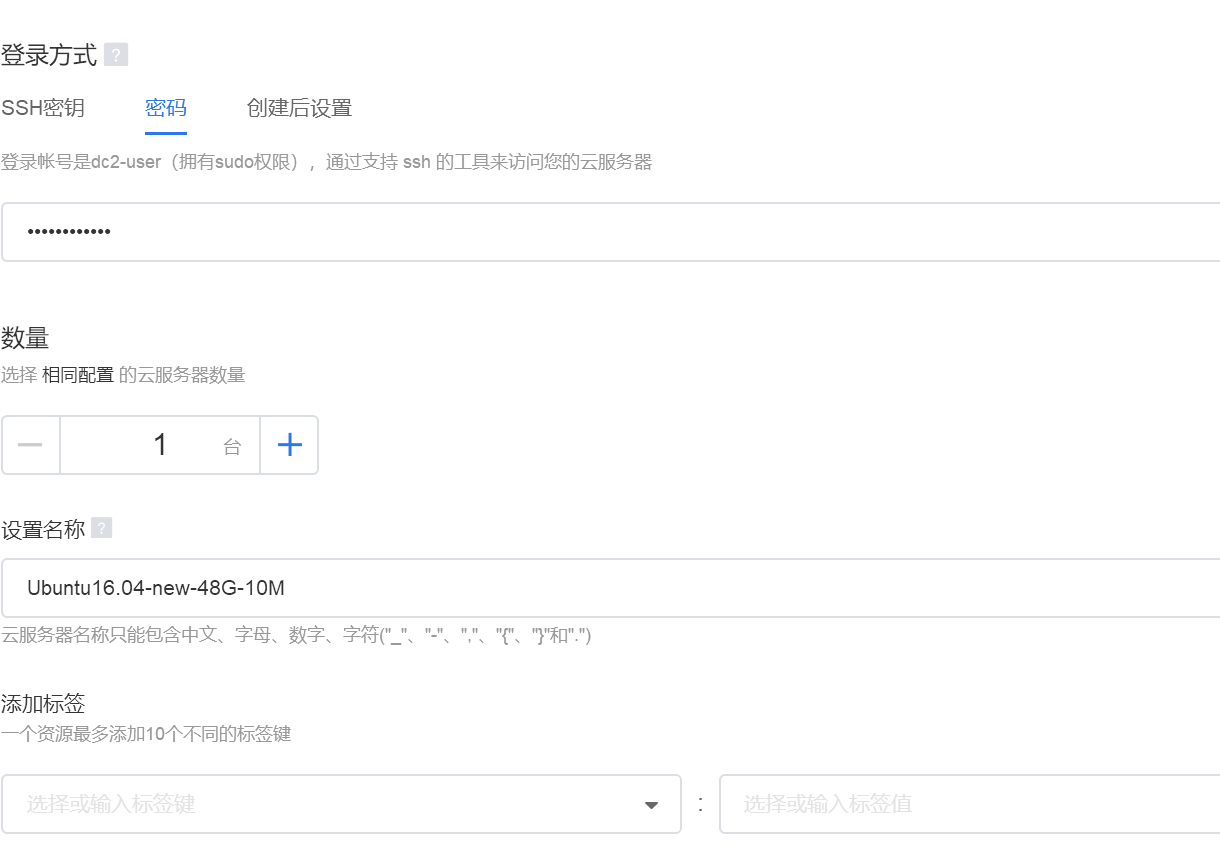创建成功，一定要记住下图中的公网IP，下面会用到。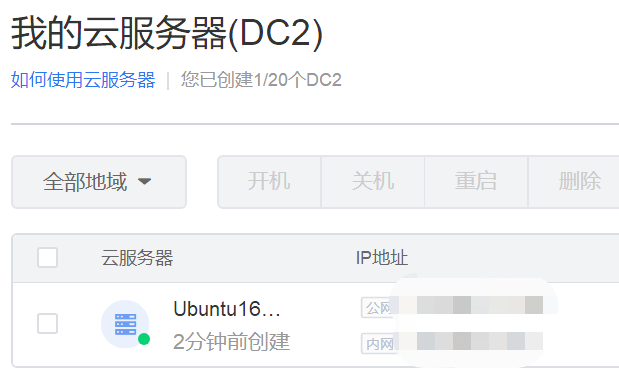## 2.远程连接

### 2.1安装WinSCP

下载WinSCP并安装。打开WinSCP，界面如下。输入上图中的公网IP地址、用户名（默认为dc2-user）和密码登陆。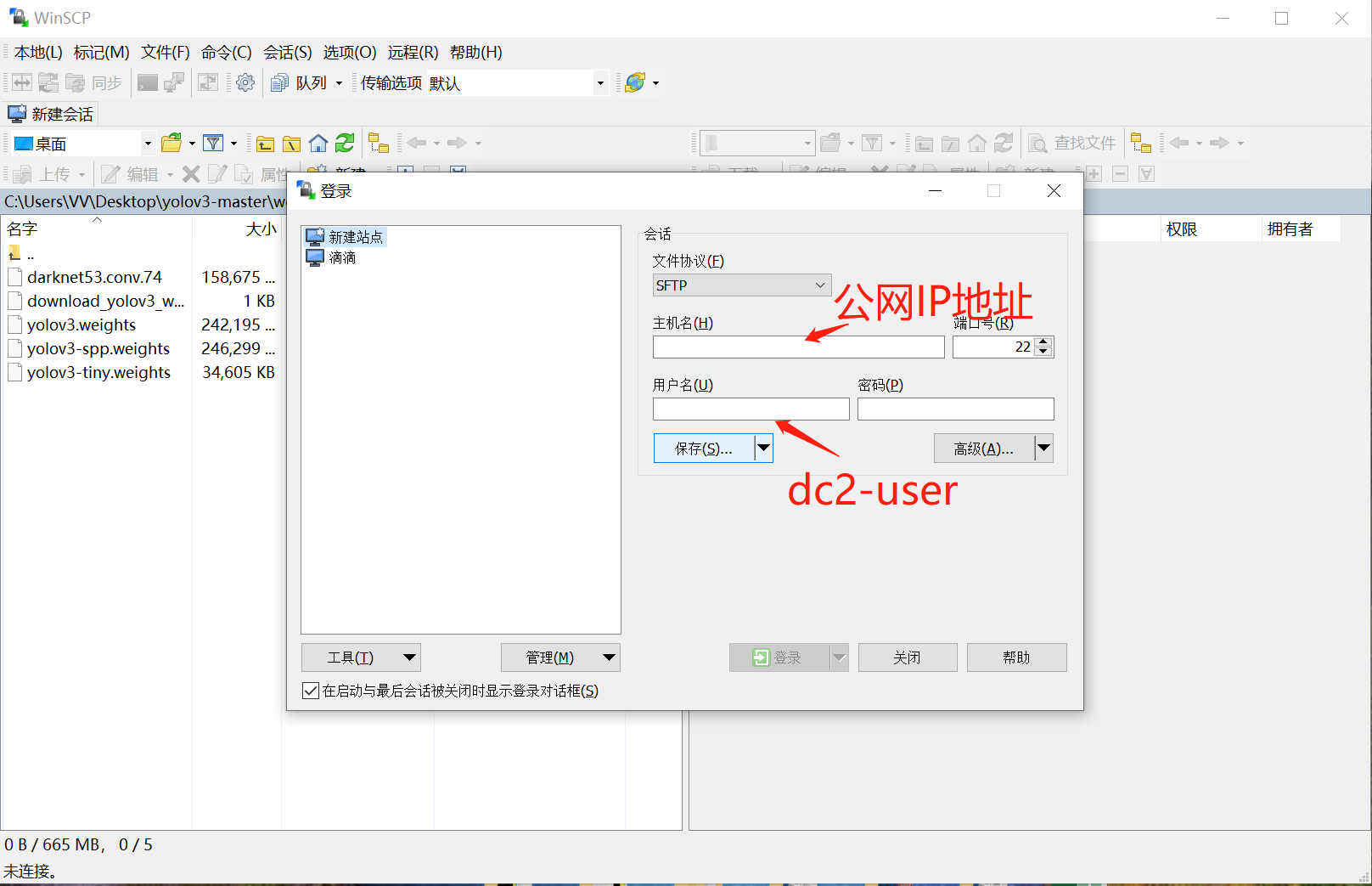为了方便以后使用，可以点击保存，即新建一个站点。然后继续登陆。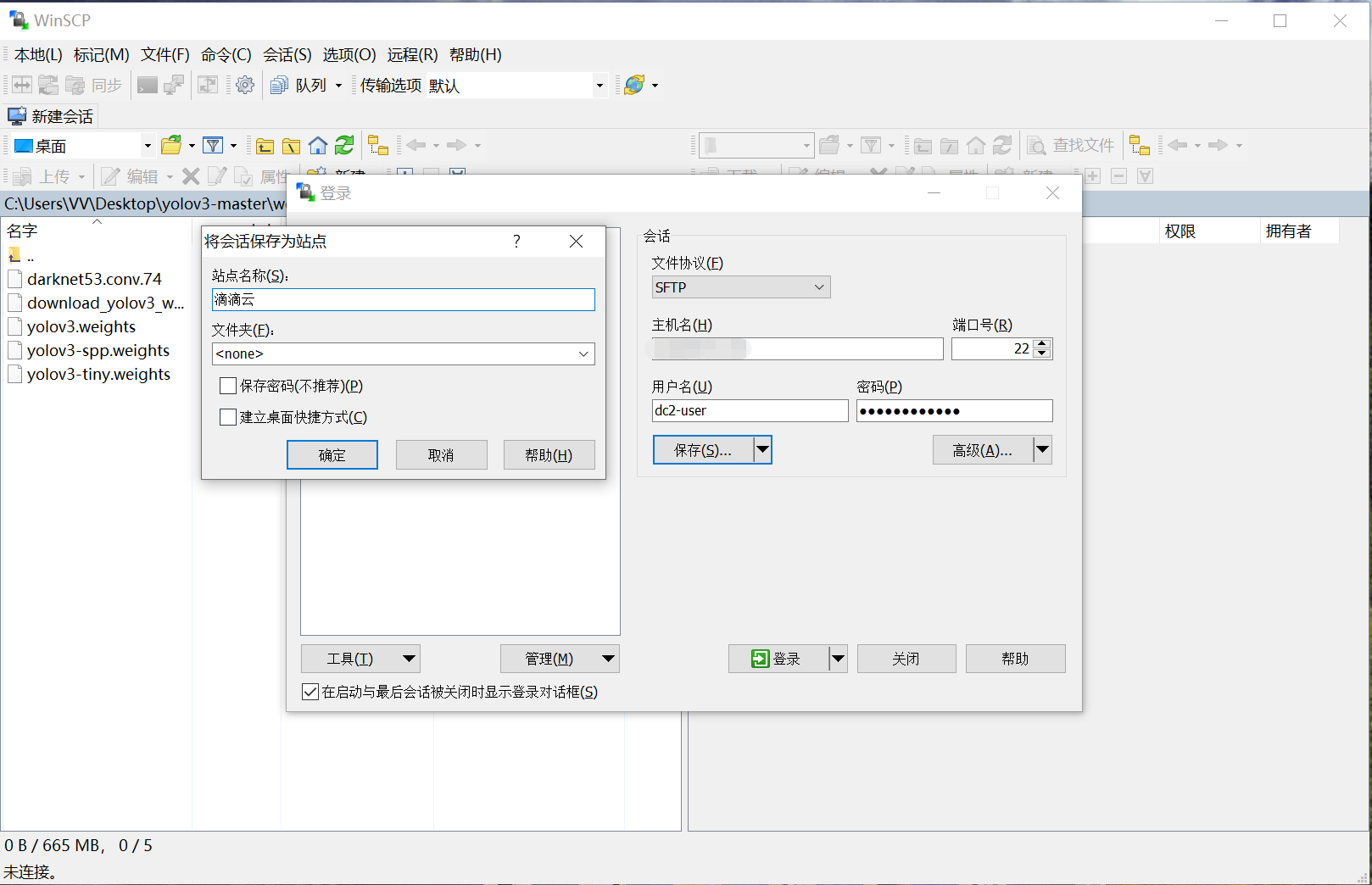点击“是”，然后会要求输入密码，以后每次只要输入密码即可。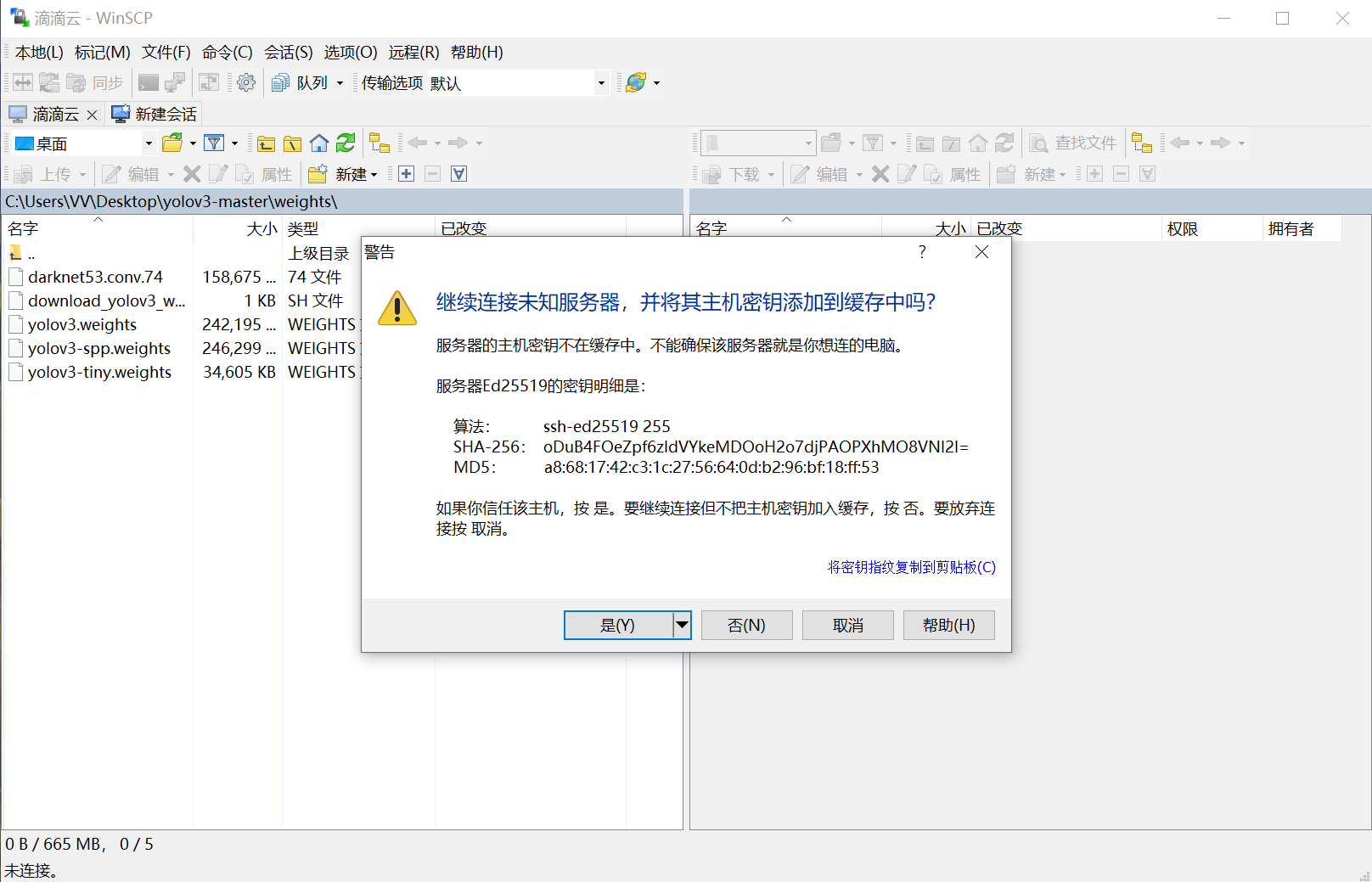右击文件，可以对文件进行操作。由于上传到服务器较慢，建议将数据集等文件压缩后再上传。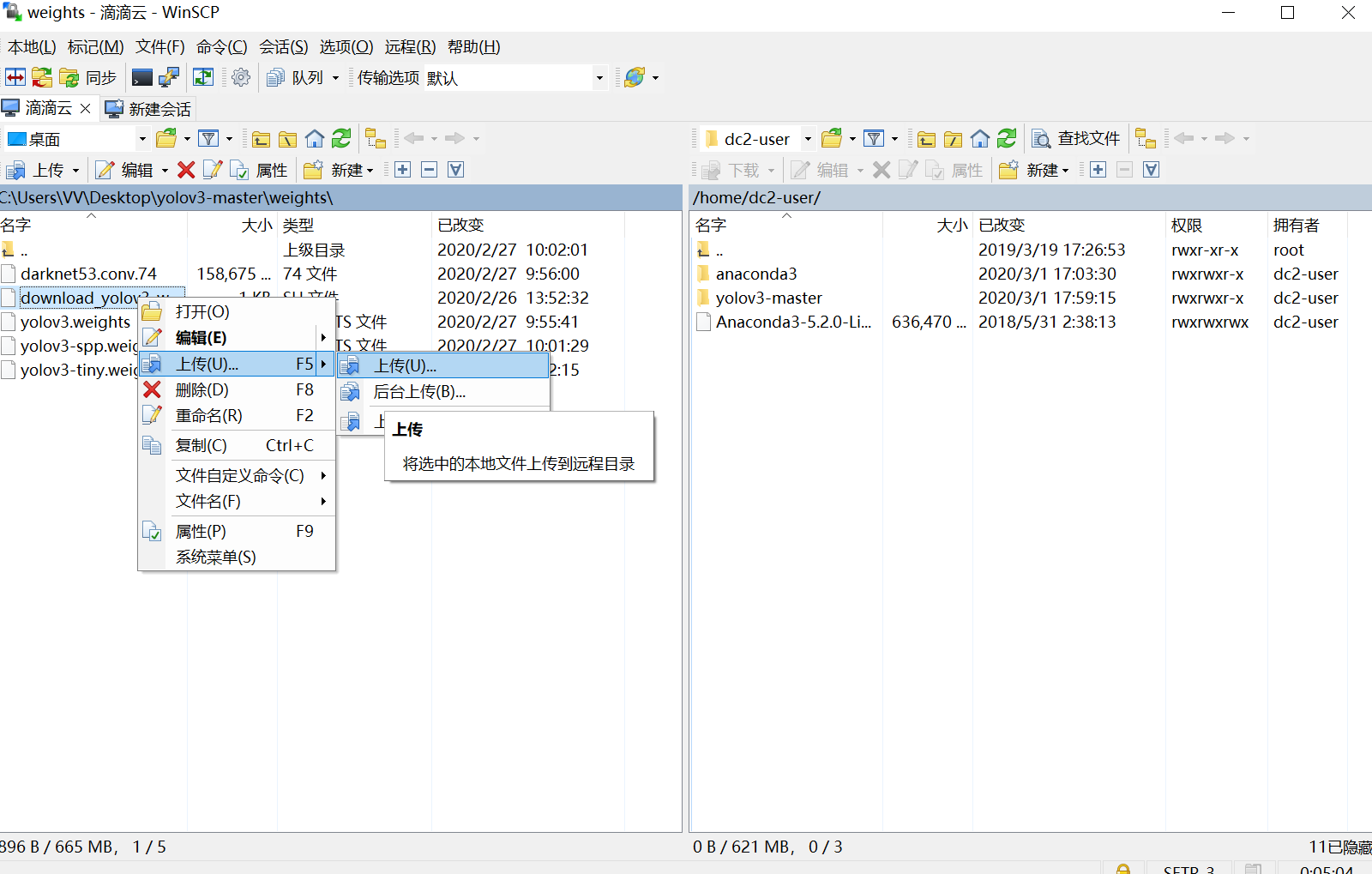### 2.2安装PuTTY

下载并安装putty，可以单独使用，也可以结合WinSCP使用。单独使用需要输入公网IP如下图所示。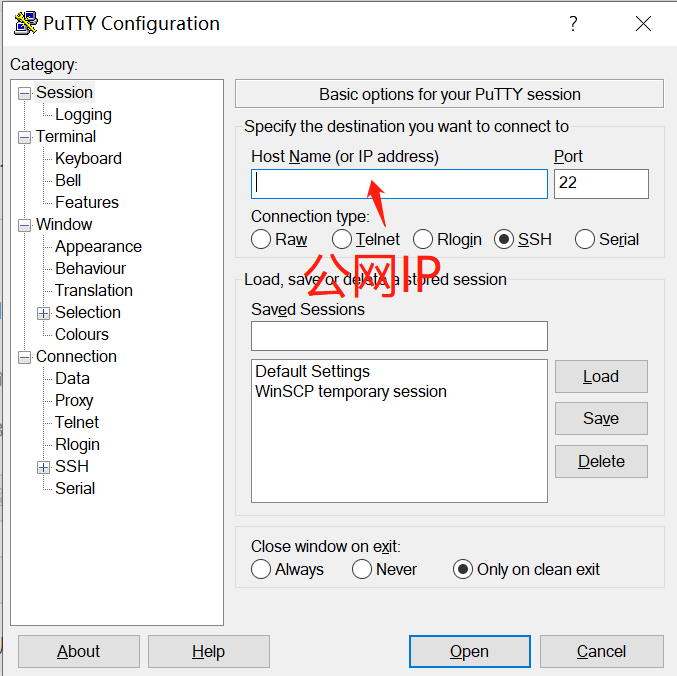或者使用 WinSCP打开，如下图所示。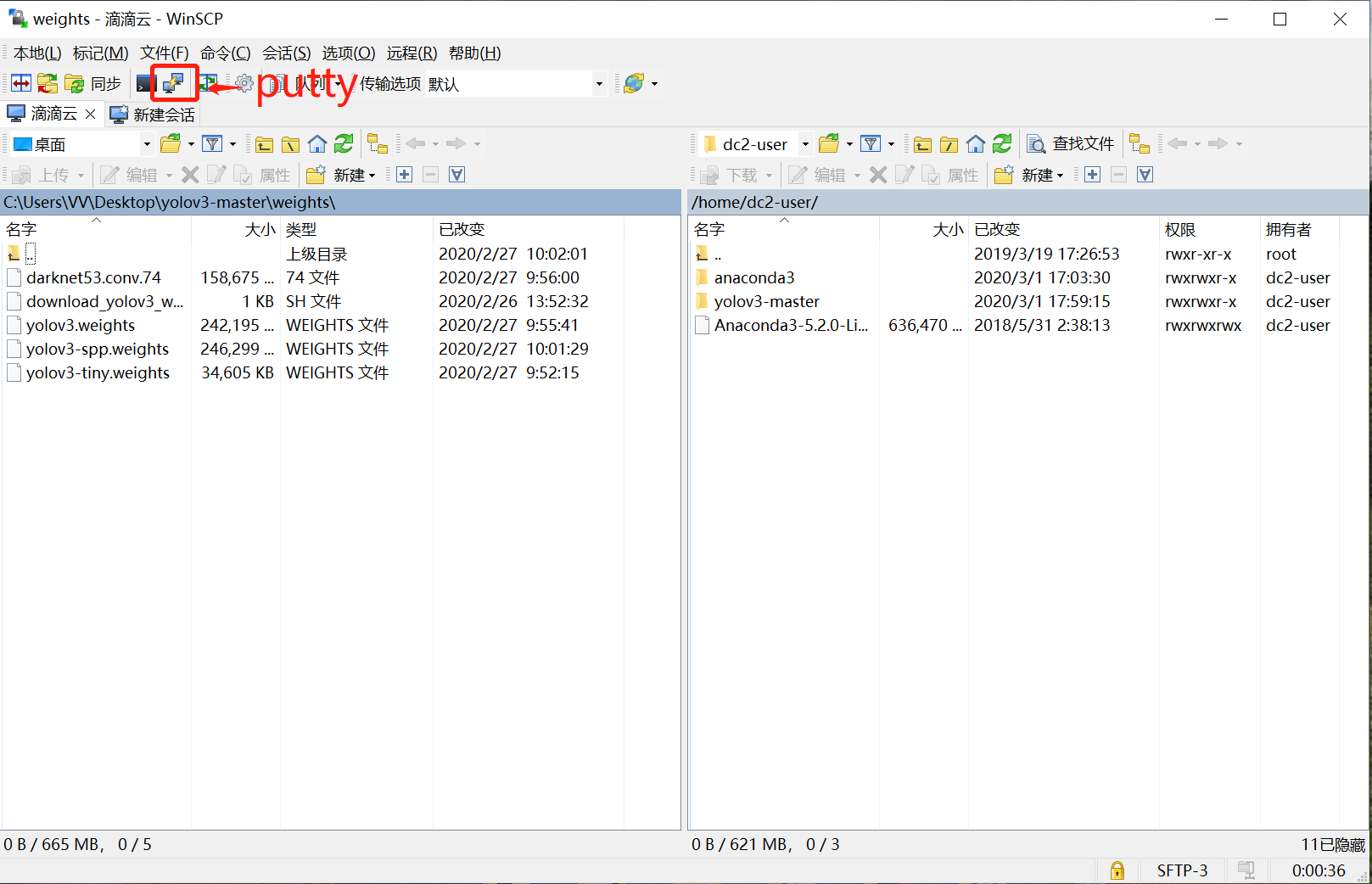这两种方法打开后界面一致，均需要输入密码。注意，密码在输入过程中无显示且界面无变化。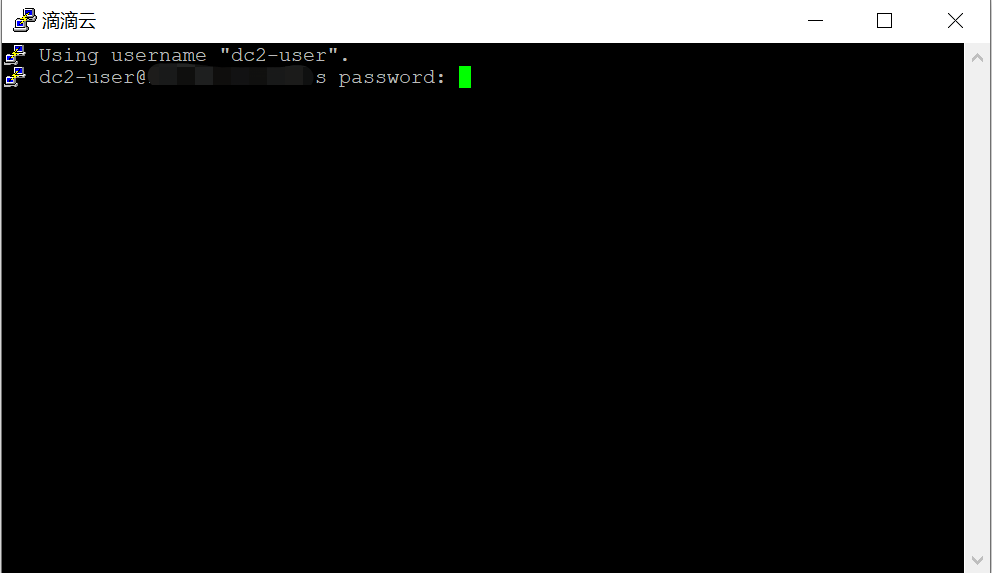输入密码，按Enter后，进入云服务器终端界面。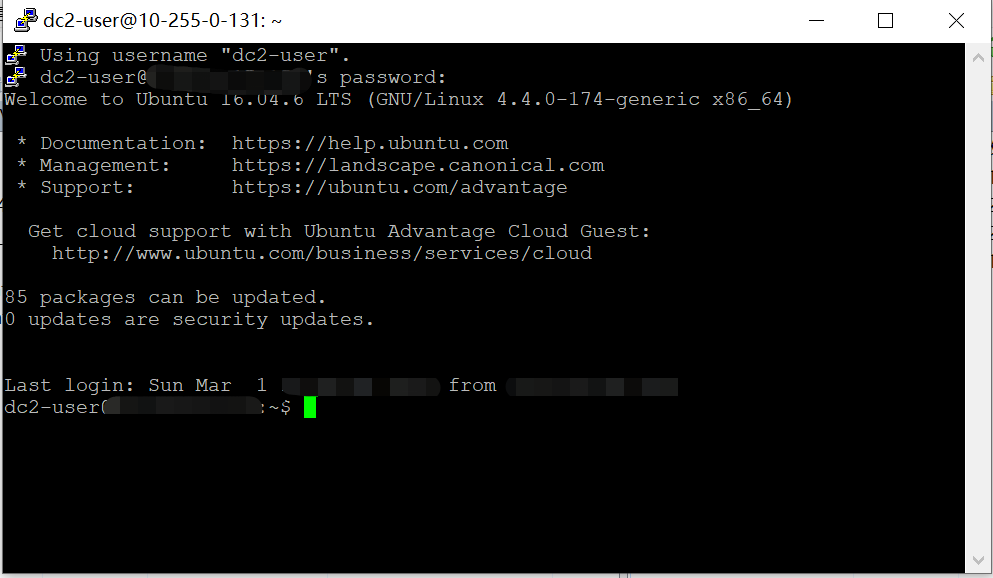下面的操作与我们平时使用的终端界面一致，输入“nvidia-smi”查看驱动和cuda信息，发现均已安装好。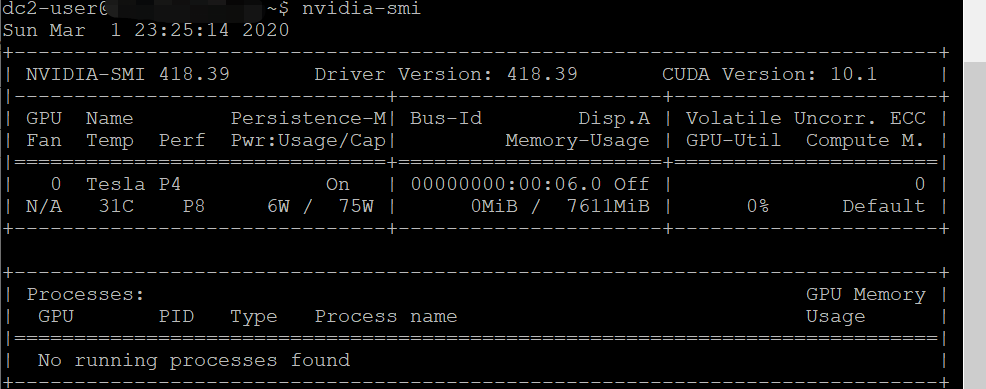安装Anaconda，运行以下命令即可。滴滴云网速很快，但是有时会不稳定。然后就可以创建虚拟环境，安装各种包了。

wget https://repo.anaconda.com/archive/Anaconda3-2019.10-Linux-x86_64.sh
chmod 777 Anaconda3-2019.10-Linux-x86_64.sh
sh Anaconda3-2019.10-Linux-x86_64.sh


安装过程一路yes，安装完成后运行

source ~/.barshrc

### 2.3 screen命令

为什么需要使用screen命令呢？因为我们在使用PuTTY时，一旦使用ctrl+d或者关闭窗口，我们的所有操作命令在云服务器都会终止。比如你在训练卷积神经网络，假如此时的电脑意外断网，云服务器就会停止训练，这是sighub信号的原因，此处不再解释。我们可以使用nohub或screen解决这个问题，screen更全面方便，所以记录screen使用流程。

在PuTTY打开的终端页面输入“screen”。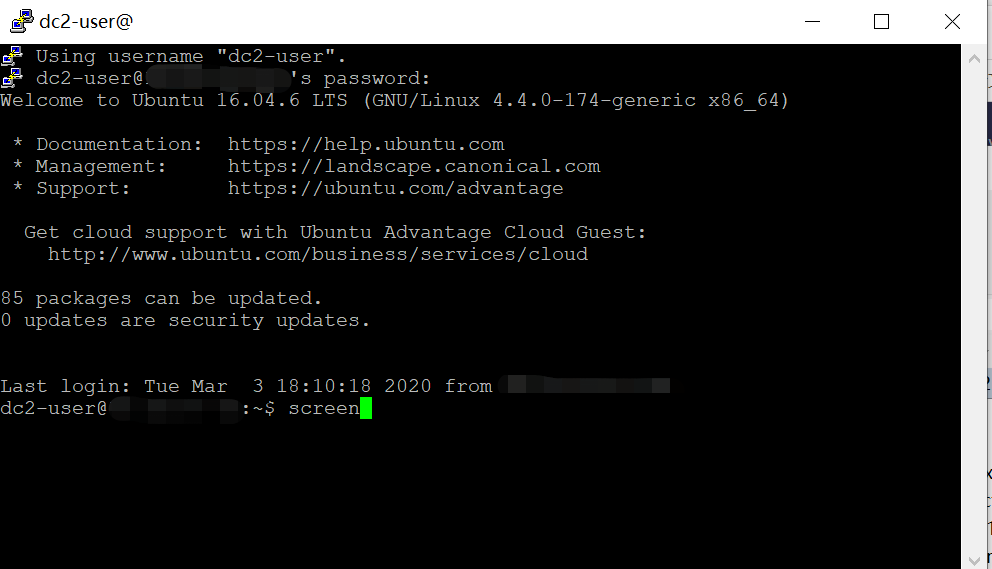出现以下界面，单击空格键继续。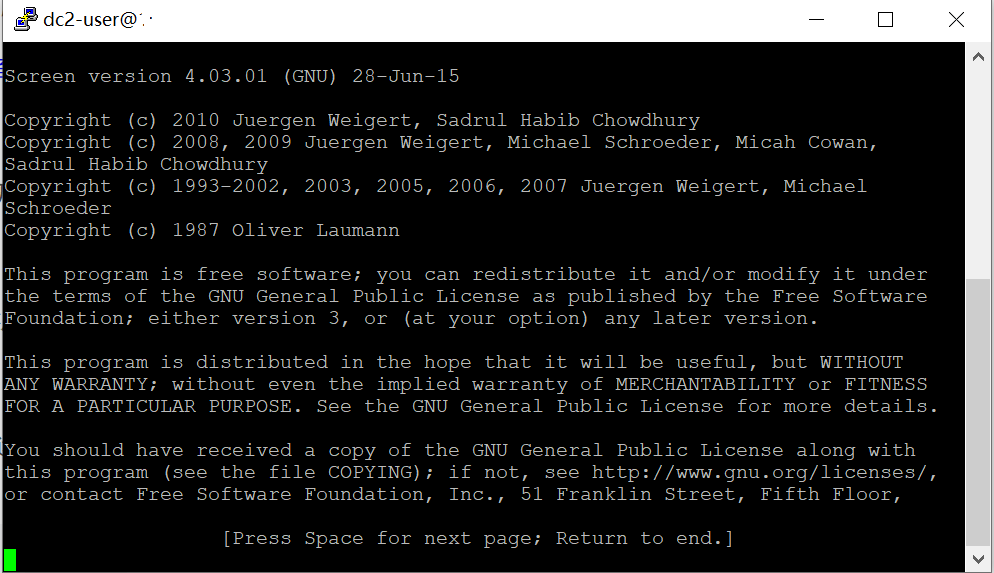然后，我们就打开了screen窗口。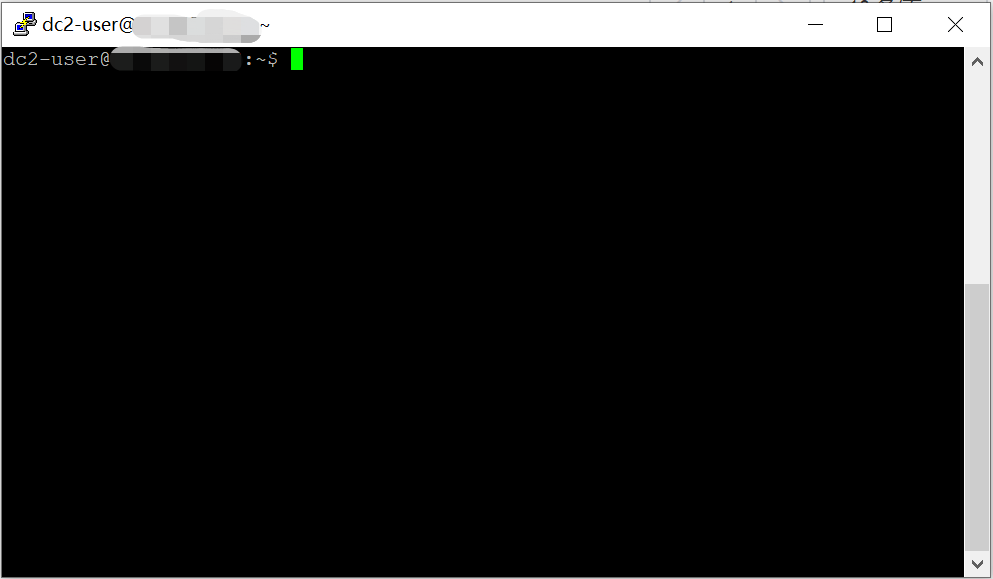输入screen -ls，可以查看当前screen窗口的代号。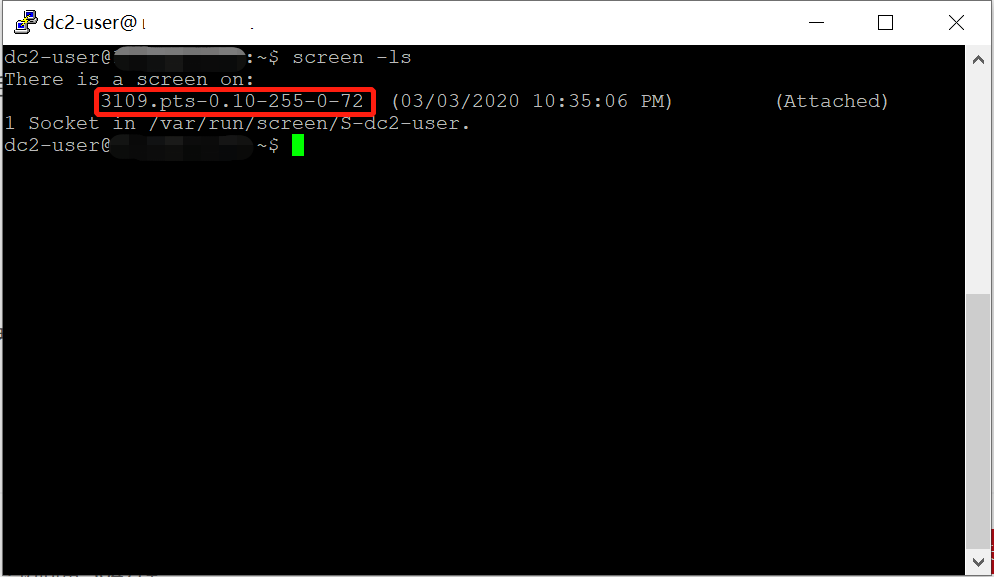先“ Ctrl+a”，然后单击“d”，即可退出screen的窗口。注意，此时仅仅是暂时退出，该screen窗口依然在运行程序。如果现在关闭PuTTY则不会影响screen里运行的程序。输入“screen -r 代号”可以重新进入screen。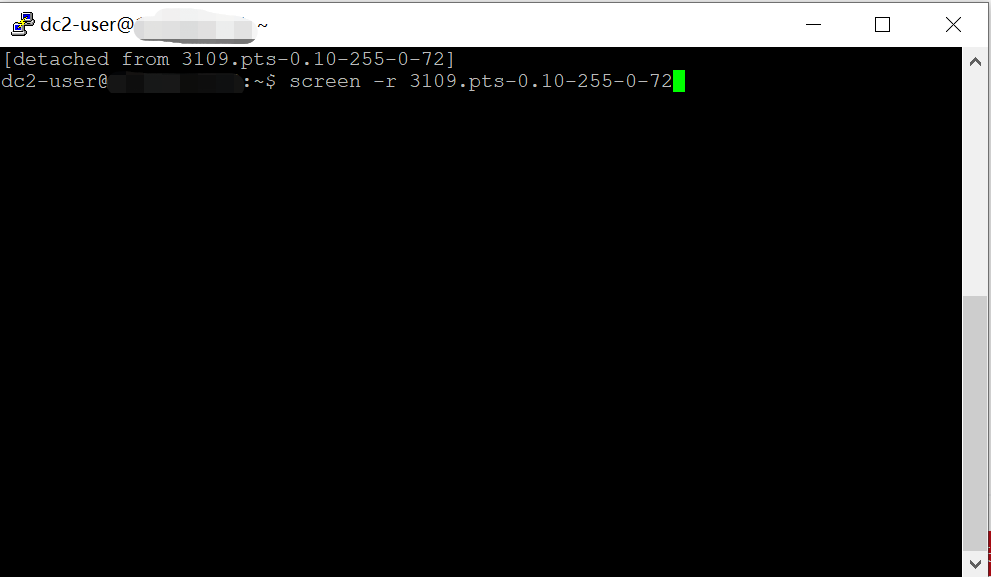先“ Ctrl+a”，然后单击“k”，即可杀死screen的窗口，真正关闭该窗口。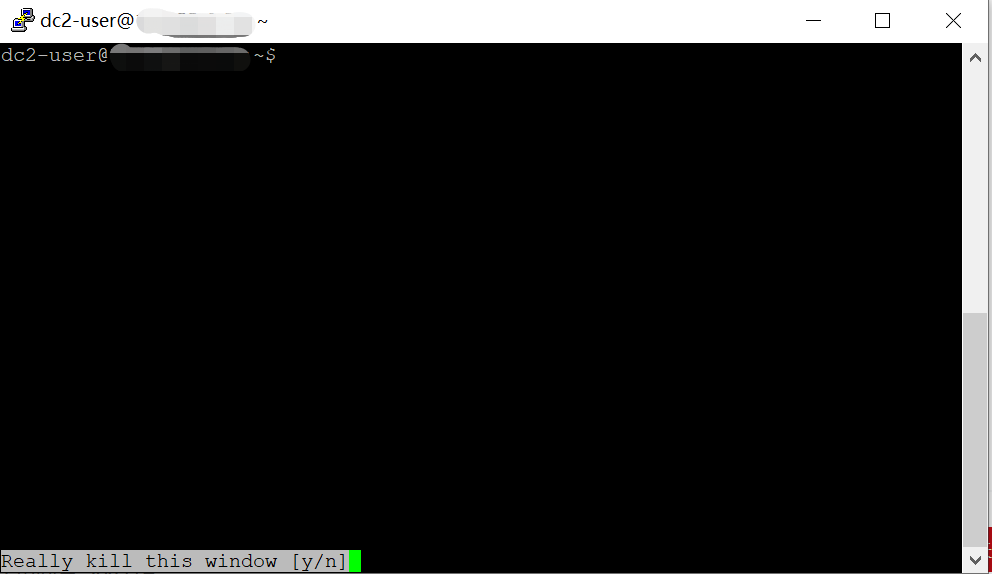下面是screen的常用键组合。

Ctrl-a ? 显示所有键绑定信息 显示所有窗口列表 切换到之前显示的窗口 创建一个新的运行shell的窗口并切换到该窗口 切换到下一个窗口 切换到前一个窗口(与C-a n相对) 切换到窗口0..9 发送 C-a到当前窗口 暂时断开screen会话 杀掉当前窗口 进入拷贝/回滚模式

## 3.快照教程

### 3.1创建快照

为什么要创建快照?因为我们不运行云服务器，云服务器也在收费，我们必须删掉实例才会停止扣费，但是我们的云服务器全都会被删除。快照相当于一个镜像，可以还原我们之前的所有工作。首先，在控制台中找到需要创建快照的云服务器，在右侧单击。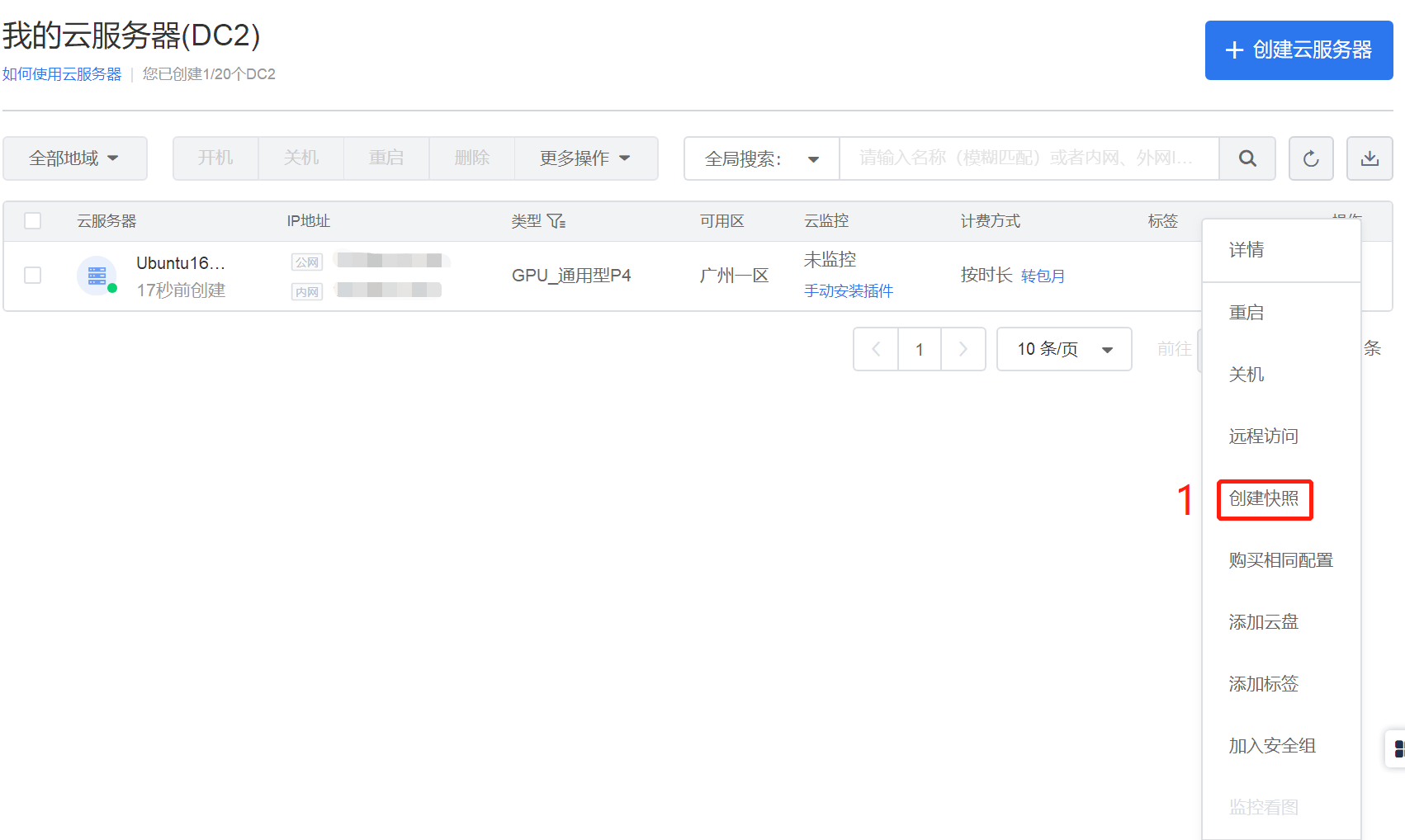### 3.2删除云服务器

创建快照后，我们就可以高枕无忧的删除云服务器了。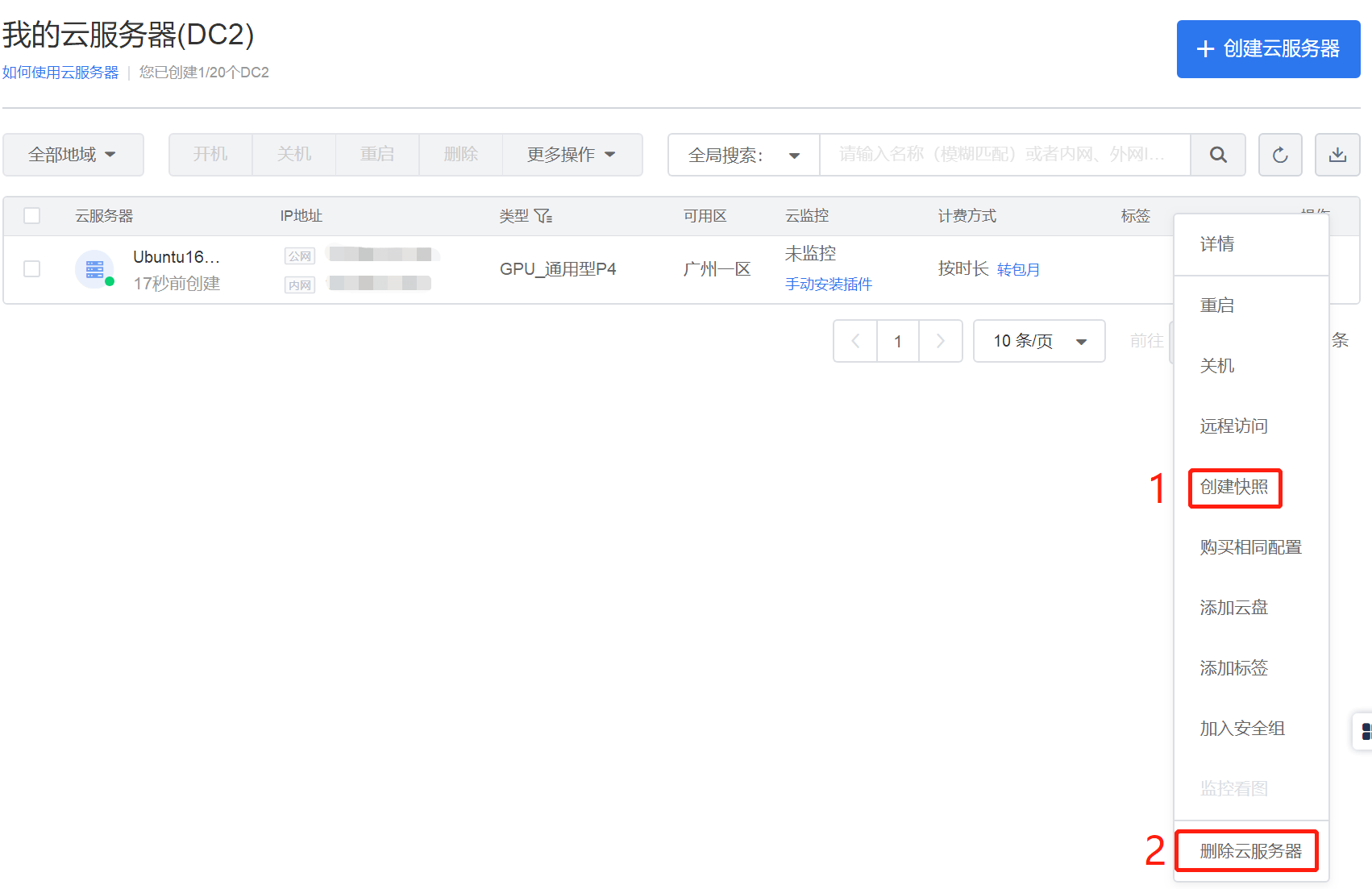### 3.3使用快照

有两种使用快照还原云服务器的方法。一种是在创建云服务器时选择快照镜像，另一种是在控制台的快照列表中找到快照新建云服务器。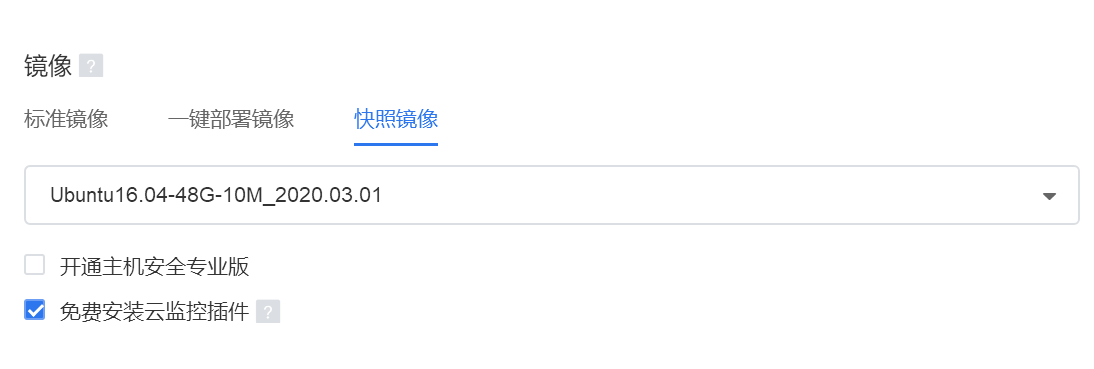云gpu 深度学习 相关内容

阅读数 1073

博文 来自： u010159842

阅读数 1477

博文 来自： u010159842
博文 来自： weixin_34161083

阅读数 18259

博文 来自： cccat6
没有更多推荐了，返回首页